# Overview

This vignette was made for version 2.0.0 of CHNOSZ, a package for the R software environment. For more information on R, see “An Introduction to R” and the contributed documentation for R.

CHNOSZ has been developed since 2006 as a tool for thermodynamic calculations in geochemistry and compositional biology. The package provides functions and a thermodynamic database that can be used to calculate the stoichiometric and energetic properties of reactions involving minerals and inorganic and/or organic aqueous species. These functions also enable calculations of chemical affinities and metastable equilibrium distributions of proteins. A major feature of the package is the production of diagrams to visualize the effects of changing temperature, pressure, and activities of basis species on the potential for reactions among various species.

After starting R, install CHNOSZ by selecting the “Install packages from CRAN” or similar menu item in the R GUI or by using the following command:

``install.packages("CHNOSZ")``

Then load the CHNOSZ package to make its data and functions available in your R session:

``library(CHNOSZ)``

CHNOSZ is now ready to go with the default thermodynamic database and an empty system definition. After running some calculations, you may want to “start over” with the default values. To clear the system settings and restore the default thermodynamic database, use `reset()`.

``reset()``
```## reset: resetting "thermo" object
```
```## OBIGT: loading default database with 1904 aqueous, 3448 total species
```

Note: Throughout this document, syntax highlighting is applied to the input of the code chunks. Double hash marks (`##`) precede the output, where black text denotes results and blue text is used for messages.

## Getting help

After CHNOSZ is installed, type `help.start()` to browse the R help documents, then choose “Packages” followed by “CHNOSZ”. That shows an index of the manual (help pages) for each function; many of the help pages include examples. There are also links to the demos (longer examples) and vignettes (more in-depth documentation; this document is a vignette).

Suggestions for accessing the documentation are indicated here with blue text. For example, read `?`CHNOSZ-package`` to get an overview of the package and a list of features. `?`” is a shortcut to R’s `help()` function. The command here is equivalent to `help(“CHNOSZ-package”)`.

## Organization of major functions

CHNOSZ is made up of a set of functions and supporting datasets. The major components of the package are shown in the figure below, which is an updated version of the diagram in Dick (2008Dick JM. 2008. Calculation of the relative metastabilities of proteins using the CHNOSZ software package. Geochemical Transactions 9: 10. doi: 10.1186/1467-4866-9-10). Rectangles and ellipses represent functions and datasets; bold text indicates primary functions.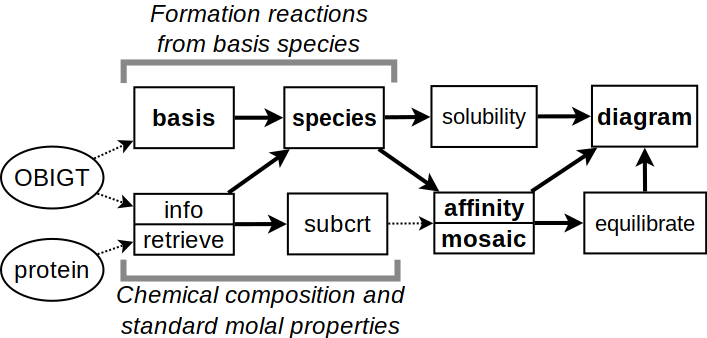Structure of CHNOSZ.

Many functions in CHNOSZ have no side effects. That is, the function only returns a result; to use the result elsewhere, it can be assigned to a variable with `<-`. In this document, the names of these functions are shown in green text (not applicable to the code chunks). When they are mentioned, names of functions in the base and recommended packages of R are said to belong to R. Example: Use R’s `plot()` to plot the data. Major functions without side effects in CHNOSZ are:

• `info()`: search for species in the thermodynamic database;
• `subcrt()`: calculate the thermodynamic properties of species and reactions;
• `affinity()`: calculate the affinities of formation reactions using given chemical activities;
• `equilibrate()`: calculate the equilibrium chemical activities of the species of interest;
• `diagram()`: plot the results.

Some functions in CHNOSZ do have side effects: they modify the `thermo` data object in the current R session. In this document, the names of these functions are shown in red text (but not in the code chunks). Major functions with side effects are:

• `basis()`: set the basis species and their chemical activities;
• `species()`: set the species of interest and their (non-equilibrium) chemical activities;
• `reset()`: reset the database, restoring all settings to their default values.

The following pseudocode shows a common sequence of commands. In actual usage, the `...` are replaced by arguments that define the chemical species and variables:

``````basis(...)
species(...)
a <- affinity(...)
e <- equilibrate(a)  ## optional
diagram(e)           ## or diagram(a)
reset()         ## clear settings for next calculation``````

# Querying the thermodynamic database

## The `info()` function

`info()` provides an interface to the OBIGT thermodynamic database that is packaged with CHNOSZ. Suppose you are interested in the thermodynamic properties of aqueous methane. Because the database is assembled with aqueous species first, they take precedence over other states. Searching by chemical formula alone gives the first matching species, in this case aqueous methane:

``info("CH4")``
```## info.character: found CH4(aq); also available in gas, liq
```
``##  884``

The number that is returned is the species index in the database. A second argument can be used to specify a physical state with lower precedence:

``info("CH4", "gas")``
``##  2650``

While some species are identified only by chemical formula, others have distinct names (in English) listed in the database. For CH4 and inorganic substances that are represented by both gaseous and aqueous forms, the name is applied only to the gas. However, the names of organic substances other than methane are applied to aqueous species, which have precedence, and those in other states. The following commands get the species indices for some common gases:

``info("methane")``
```## info.character: found methane(gas); also available in liq
```
``##  2650``
``info("oxygen")``
``##  2641``
``info("carbon dioxide")``
``##  2633``

A special case is sulfur; the name refers to both the native mineral, which has precedence, and the gas. These two phases can be identifed with the formulas S2 and S, respectively.

``info("S")``
```## info.character: found S(cr) [sulfur] with 2 phase transitions
```
``##  1993``
``info("S2")``
``##  2643``

Taking the species number of aqueous methane returned by `info()`, use the function again to retrieve the set of standard molal thermodynamic properties and equations of state parameters:

``````iCH4 <- info("CH4")
info(iCH4)``````
``````##     name abbrv formula state ref1 ref2       date model E_units     G      H  S
## 884  CH4  <NA>     CH4    aq PS01 <NA> 2000-10-04   HKF     cal -8140 -20930 21
##        Cp  V    a1    a2     a3     a4    c1    c2  omega Z
## 884 60.23 36 1.769 -1530 -67.88 114700 40.87 64500 -40000 0``````

Liquid water is species number 1; it has NA entries in the database because dedicated functions are used to compute its properties:

``info(info("water"))``
``````##    name abbrv formula state  ref1  ref2       date model E_units  G  H  S Cp  V
## 1 water  <NA>     H2O   liq HGK84 JOH92 2006-10-25   H2O     cal NA NA NA NA NA
##    a  b  c  d  e  f lambda  T
## 1 NA NA NA NA NA NA     NA NA``````

## Fuzzy searches

Calling `info()` with a string that does not exactly match the name of any species invokes a fuzzy search of the database:

``info("acid")``
```## info.approx: 'acid' is ambiguous; has approximate matches to 86 species:
```
```##   "a-aminobutyric acid"         "acetamide"                   "formic acid"                 "acetic acid"                 "propanoic acid"
##   "n-butanoic acid"             "n-pentanoic acid"            "n-hexanoic acid"             "n-heptanoic acid"            "n-octanoic acid"
##  "n-nonanoic acid"             "n-decanoic acid"             "n-undecanoic acid"           "n-dodecanoic acid"           "n-benzoic acid"
##  "o-toluic acid"               "m-toluic acid"               "p-toluic acid"               "oxalic acid"                 "malonic acid"
##  "succinic acid"               "glutaric acid"               "adipic acid"                 "pimelic acid"                "suberic acid"
##  "azelaic acid"                "sebacic acid"                "glycolic acid"               "lactic acid"                 "2-hydroxybutanoic acid"
##  "2-hydroxypentanoic acid"     "2-hydroxyhexanoic acid"      "2-hydroxyheptanoic acid"     "2-hydroxyoctanoic acid"      "2-hydroxynonanoic acid"
##  "2-hydroxydecanoic acid"      "uracil"                      "citric acid"                 "aspartic acid"               "glutamic acid"
##  "cis-aconitic acid"           "isocitric acid"              "a-ketoglutaric acid"         "fumaric acid"                "malic acid"
##  "oxaloacetic acid"            "pyruvic acid"                "cyclohexane carboxylic acid" "N-acetylmuramic acid"        "diaminopimelic acid"
##  "metacinnabar"                "acide fulvique"              "acide humique"               "aspartic acid"               "glutamic acid"
##  "2-iodobenzoic acid"          "3-iodobenzoic acid"          "4-iodobenzoic acid"          "uracil"                      "phosphoric acid"
##  "citric acid"                 "acetamide"                   "nicotinamide,red"            "nicotinamide,ox"             "2-iodobenzoic acid"
##  "3-iodobenzoic acid"          "4-iodobenzoic acid"          "acetic acid"                 "propanoic acid"              "n-butanoic acid"
##  "n-pentanoic acid"            "n-hexanoic acid"             "n-heptanoic acid"            "n-octanoic acid"             "n-nonanoic acid"
##  "n-decanoic acid"             "n-undecanoic acid"           "n-dodecanoic acid"           "n-tridecanoic acid"          "n-tetradecanoic acid"
##  "n-eicosanoic acid"
```
``##  NA``

The message includes e.g. “uracil” and “metacinnabar” because their names have some similarity to the search term. Since “ribose” is the name of a species in the database, to find species with similar names, add an extra character to the search:

``info(" ribose")``
```## info.approx: ' ribose' is ambiguous; has approximate matches to 8 species:
```
```##  "ribose"               "deoxyribose"          "ribose-5-phosphate"
##  "ribose-5-phosphate-1" "ribose-5-phosphate-2" "ribose"
##  "deoxyribose"          "ribose-5-phosphate"
```
``##  NA``

The messages may be useful for browsing the database, but owing to their ambiguous results, these fuzzy searches return an `NA` value for the species index.

## Counting elements, chemical formulas, `ZC()`

Continuing with the example of aqueous methane, let’s look at its chemical formula:

``info(iCH4)\$formula``
``##  "CH4"``

We can use `makeup()` to count the elements in the formula, followed by `as.chemical.formula()` to rewrite the formula on one line:

``makeup(iCH4)``
``````## C H
## 1 4``````
``as.chemical.formula(makeup(iCH4))``
``##  "CH4"``

For organic species, a calculation of the average oxidation state of carbon (ZC) is possible given the species index, chemical formula, or elemental count:

``ZC(iCH4)``
``##  -4``
``ZC(info(iCH4)\$formula)``
``##  -4``
``ZC(makeup(iCH4))``
``##  -4``

# Calculating thermodynamic properties

To calculate the standard molal properties of species and reactions, use `subcrt()`. The name of this function is derived from the SUPCRT package (Johnson et al., 1992Johnson JW, Oelkers EH, Helgeson HC. 1992. SUPCRT92: A software package for calculating the standard molal thermodynamic properties of minerals, gases, aqueous species, and reactions from 1 to 5000 bar and 0 to 1000°C. Computers & Geosciences 18(7): 899–947. doi: 10.1016/0098-3004(92)90029-Q), which is also the source of the Fortran subroutine used to calculate the thermodynamic properties of H2O.

If no reaction coefficients are given, `subcrt()` calculates the standard molal properties of individual species:

``subcrt("water")``
```## subcrt: 1 species at 15 values of T (ºC) and P (bar) (wet) [energy units: J]
```
``````## \$species
##    name formula state ispecies          model
## 1 water     H2O   liq        1 water.SUPCRT92
##
## \$out
## \$out\$water
##         T         P      rho    logK       G       H        S       V       Cp
## 1    0.01   1.00000 0.999829 45.0353 -235515 -287724  63.3139 18.0183  76.1722
## 2   25.00   1.00000 0.997061 41.5525 -237181 -285837  69.9242 18.0683  75.3605
## 3   50.00   1.00000 0.988030 38.6328 -239006 -283954  75.9912 18.2335  75.3314
## 4   75.00   1.00000 0.974864 36.1544 -240977 -282069  81.6083 18.4797  75.4862
## 5  100.00   1.01322 0.958393 34.0270 -243084 -280176  86.8580 18.7973  75.9728
## 6  125.00   2.32014 0.939073 32.1831 -245315 -278267  91.8050 19.1840  76.7067
## 7  150.00   4.75717 0.917058 30.5718 -247665 -276335  96.4997 19.6446  77.6819
## 8  175.00   8.91805 0.892343 29.1531 -250125 -274373 100.9851 20.1887  79.0063
## 9  200.00  15.53650 0.864743 27.8960 -252691 -272370 105.3036 20.8330  80.8718
## 10 225.00  25.47860 0.833873 26.7753 -255355 -270311 109.5002 21.6042  83.5561
## 11 250.00  39.73649 0.799072 25.7711 -258112 -268173 113.6247 22.5452  87.4971
## 12 275.00  59.43125 0.759236 24.8670 -260959 -265925 117.7377 23.7281  93.5177
## 13 300.00  85.83784 0.712408 24.0494 -263890 -263512 121.9248 25.2878 103.5098
## 14 325.00 120.45757 0.654577 23.3072 -266901 -260836 126.3367 27.5219 123.2082
## 15 350.00 165.21129 0.574688 22.6310 -269989 -257632 131.3656 31.3478 182.4162``````

That uses the default temperature and pressure settings, i.e. equally spaced temperature intervals from 0 to 350 °C at Psat, i.e. 1 bar below 100 °C, or the pressure of liquid-vapor saturation (i.e. boiling) at higher temperatures. The columns in the output are temperature, pressure, density of water, logarithm of the equilibrium constant (only meaningful for reactions; see below), standard molal Gibbs energy and enthalpy of formation from the elements, standard molal entropy, volume, and heat capacity. The corresponding units are °C (`T`), bar (`P`), g cm-3 (`rho`), cal mol-1 (`G` and `H`), cal K-1 mol-1 (`S` and `Cp`), and cm3 mol-1 (`V`).

A custom temperature-pressure grid can be specified. Here, we calculate the properties of H2O on a T, P grid in the supercritical region, with conditions grouped by pressure:

``subcrt("water", T = c(400, 500, 600), P = c(200, 400, 600), grid = "P")\$out\$water``
```## subcrt: 1 species at 9 values of T (ºC) and P (bar) (wet) [energy units: J]
```
``````##     T   P       rho    logK       G       H       S        V       Cp
## 1 400 200 0.1005447 21.5271 -277426 -236979 163.338 179.1760 114.7691
## 2 500 200 0.0677109 19.8868 -294360 -229366 173.961 266.0607  58.8996
## 3 600 200 0.0550386 18.6704 -312097 -224011 180.484 327.3197  50.0435
## 4 400 400 0.5236661 21.4119 -275941 -252941 137.419  34.4021 157.0325
## 5 500 400 0.1779739 19.6639 -291060 -235360 161.939 101.2238 104.4640
## 6 600 400 0.1238075 18.4124 -307785 -227449 171.608 145.5098  64.8105
## 7 400 600 0.6124511 21.3631 -275313 -254523 134.136  29.4149 108.2861
## 8 500 600 0.3384430 19.5660 -289610 -241392 152.263  53.2296 135.7227
## 9 600 600 0.2071976 18.2784 -305546 -230936 165.050  86.9469  81.0704``````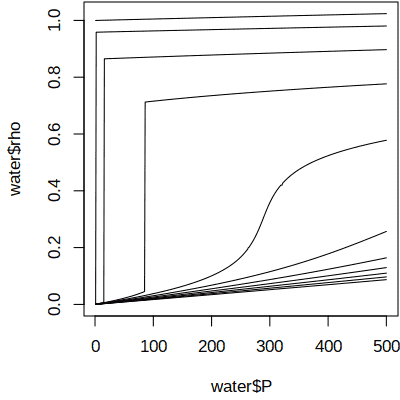Isothermal contours of density (g cm-3) and pressure (bar) of water.

The additional operations (`\$out\$water`) are used to extract a specific part of the results; this can be used with e.g. R’s `write.table()` or `plot()` for further processing:

``````sres <- subcrt("water", T=seq(0,1000,100), P=c(NA, seq(1,500,1)), grid="T")
water <- sres\$out\$water
plot(water\$P, water\$rho, type = "l")``````

## Changing units

The default units of temperature, pressure, and energy in the input and output of `subcrt()` are °C, bar, and Joules. The functions `T.units()`, `P.units()`, and `E.units()` can be used to change the units used by `subcrt()` and some other functions in CHNOSZ. What is the Gibbs energy in J/mol (the default) and cal/mol of aqueous methane at 298.15 K and 0.1 MPa?

``T.units("K")``
```## changed temperature units to K
```
``P.units("MPa")``
```## changed pressure units to MPa
```
``subcrt("CH4", T = 298.15, P = 0.1)\$out\$CH4\$G``
```## info.character: found CH4(aq); also available in gas, liq
```
```## subcrt: 1 species at 298.15 K and 0.1 MPa (wet) [energy units: J]
```
``##  -34057.8``
``E.units("cal")``
```## changed energy units to cal
```
``subcrt("CH4", T = 298.15, P = 0.1)\$out\$CH4\$G``
```## info.character: found CH4(aq); also available in gas, liq
```
```## subcrt: 1 species at 298.15 K and 0.1 MPa (wet) [energy units: cal]
```
``##  -8140``

The parameters for each species in the OBIGT database have the units indicated by the `E_units` column there, which is independent of the setting of `E.units()`. Unlike `subcrt()`, `info()` always displays the database values in the units given in the database. A separate function, `convert()`, can be used to convert values to selected units. The following code shows that the database parameters for CH4(aq) are in calories, then converts the standard Gibbs energy from cal/mol to J/mol.

``(CH4dat <- info(info("CH4")))``
``````##     name abbrv formula state ref1 ref2       date model E_units     G      H  S
## 884  CH4  <NA>     CH4    aq PS01 <NA> 2000-10-04   HKF     cal -8140 -20930 21
##        Cp  V    a1    a2     a3     a4    c1    c2  omega Z
## 884 60.23 36 1.769 -1530 -67.88 114700 40.87 64500 -40000 0``````
``convert(CH4dat\$G, "J")``
``##  -34057.8``

Note that converting the database value to J/mol gives the same result as running `subcrt("CH4", T = 25)` with the default `E.units()` setting of J.

Use `reset()` to restore the units and all other settings for CHNOSZ to their defaults:

``reset()``
```## reset: resetting "thermo" object
```
```## OBIGT: loading default database with 1904 aqueous, 3448 total species
```

# Properties of reactions

## Reaction definitions

To calculate the thermodynamic properties of reactions, give the names of species, the physical states (optional), and reaction coefficients as the arguments to `subcrt()`. Here we calculate properties for the dissolution of CO2. This doesn’t correspond to the solubility of CO2; see the solubility section in this vignette and `demo(solubility)` for examples of solubility calculations.

``subcrt(c("CO2", "CO2"), c("gas", "aq"), c(-1, 1), T = seq(0, 250, 50))``
```## subcrt: 2 species at 6 values of T (ºC) and P (bar) (wet) [energy units: J]
```
``````## \$reaction
##      coeff           name formula state ispecies model
## 2633    -1 carbon dioxide     CO2   gas     2633   CGL
## 707      1            CO2     CO2    aq      707   HKF
##
## \$out
##        T        P      rho     logK        G         H         S       V
## 1   0.01  1.00000 0.999829 -1.10873  5798.19 -24718.86 -111.7182 23.6978
## 2  50.00  1.00000 0.988030 -1.71865 10632.66 -16517.28  -84.0162 36.9843
## 3 100.00  1.01322 0.958393 -2.00354 14313.00  -9506.03  -63.8321 41.5584
## 4 150.00  4.75717 0.917058 -2.10770 17074.67  -2494.13  -46.2453 44.5642
## 5 200.00 15.53650 0.864743 -2.09920 19015.19   5141.36  -29.3221 47.9553
## 6 250.00 39.73649 0.799072 -2.01184 20149.75  14698.81  -10.4193 54.3882
##        Cp
## 1 205.626
## 2 144.875
## 3 138.259
## 4 143.687
## 5 164.790
## 6 233.124``````

In order to make a plot like Figure 18 of Manning et al. (2013Manning CE, Shock EL, Sverjensky DA. 2013. The chemistry of carbon in aqueous fluids at crustal and upper-mantle conditions: Experimental and theoretical constraints. Reviews in Mineralogy and Geochemistry 75(1): 109–148. doi: 10.2138/rmg.2013.75.5), let’s run more calculations and store the results. In addition to the reaction definition, we specify a greater number of temperature points than the default: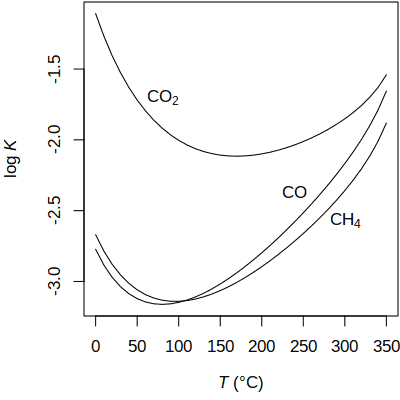Calculated equilibrium constants for dissolution of CO2, CO, and CH4.

``````T <- seq(0, 350, 10)
CO2 <- subcrt(c("CO2", "CO2"), c("gas", "aq"), c(-1, 1), T = T)\$out\$logK
CO <- subcrt(c("CO", "CO"), c("gas", "aq"), c(-1, 1), T = T)\$out\$logK
CH4 <- subcrt(c("CH4", "CH4"), c("gas", "aq"), c(-1, 1), T = T)\$out\$logK
logK <- data.frame(T, CO2, CO, CH4)``````

Now we can make the plot, using R’s `matplot()`. Here, `axis.label()` and `expr.species()` are used to create formatted axis labels and chemical formulas:

``````matplot(logK[, 1], logK[, -1], type = "l", col = 1, lty = 1,
xlab = axis.label("T"), ylab = axis.label("logK"))
text(80, -1.7, expr.species("CO2"))
text(240, -2.37, expr.species("CO"))
text(300, -2.57, expr.species("CH4"))``````

## Unbalanced reactions

A balanced chemical reaction conserves mass. `subcrt()` won’t stop you from running an unbalanced reaction, but it will give you a warning:

``subcrt(c("CO2", "CH4"), c(-1, 1))``
```## info.character: found CO2(aq); also available in gas
```
```## info.character: found CH4(aq); also available in gas, liq
```
```## subcrt: 2 species at 15 values of T (ºC) and P (bar) (wet) [energy units: J]
```
```## subcrt: reaction is not balanced; it is missing this composition:
```
```##  H  O
## -4  2
```
```## Warning in subcrt(c("CO2", "CH4"), c(-1, 1)): reaction among CO2,CH4 was
## unbalanced, missing H-4O2
```

In other words, to balance the reaction, we should add 4 H to the left and 2 O to the right. That could be done manually be redefining the reaction with the appropriate species. There is another option: balancing the reaction automatically using basis species.

## Setting the basis species

Basis species are a minimal number of chemical species that linearly combine to give the composition of any species in the system. The basis species are similar to thermodynamic components, but can include charged species. Basis species are used in CHNOSZ to automatically balance reactions; they are also required for making chemical activity diagrams.

``basis(c("CO2", "H2", "H2CO2"))``
```## Error in put.basis(ispecies, logact): singular stoichiometric matrix
```

That set of species has a singular (non-invertible) stoichiometric matrix. An error would also result from either an underdetermined or overdetermined system. A valid set of basis species has an invertible stoichiometric matrix and the same number of species as elements:

``basis(c("CO2", "H2", "H2O"))``
``````##     C H O ispecies logact state
## CO2 1 0 2      707      0    aq
## H2  0 2 0       62      0    aq
## H2O 0 2 1        1      0   liq``````

The composition of any species made up of C, H, and O can be represented by a single linear combination of these basis species.

## Automatically balancing reactions

Methanogenic metabolism in reducing environments may proceed by acetoclastic or hydrogenotrophic processes. To consider reactions involving a charged species (acetate), let’s define a basis with H+:

``basis(c("CO2", "H2", "H2O", "H+"))``
``````##     C H O Z ispecies logact state
## CO2 1 0 2 0      707      0    aq
## H2  0 2 0 0       62      0    aq
## H2O 0 2 1 0        1      0   liq
## H+  0 1 0 1        3      0    aq``````

By identifying species other than the basis species, the reactions will be automatically balanced. This produces the balanced reaction for acetoclastic methanogenesis:

``subcrt(c("acetate", "CH4"), c(-1, 1))\$reaction``
``````##      coeff    name formula state ispecies model
## 1082    -1 acetate C2H3O2-    aq     1082   HKF
## 884      1     CH4     CH4    aq      884   HKF
## 707      1     CO2     CO2    aq      707   HKF
## 3       -1      H+      H+    aq        3   HKF``````

We can similarly consider reactions for hydrogenotrophic methanogenesis as well as acetate oxidation (without production of methane):

``````acetate_oxidation <- subcrt("acetate", -1)
hydrogenotrophic <- subcrt("CH4", 1)
acetoclastic <- subcrt(c("acetate", "CH4"), c(-1, 1))``````

Use `describe.reaction()` to write the reactions on a plot: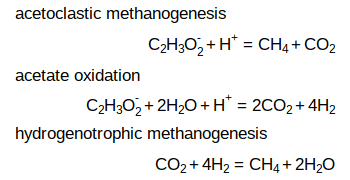``````plot(0, 0, type = "n", axes = FALSE, ann=FALSE, xlim=c(0, 5), ylim=c(5.2, -0.2))
text(0, 0, "acetoclastic methanogenesis", adj = 0)
text(5, 1, describe.reaction(acetoclastic\$reaction), adj = 1)
text(0, 2, "acetate oxidation", adj = 0)
text(5, 3, describe.reaction(acetate_oxidation\$reaction), adj = 1)
text(0, 4, "hydrogenotrophic methanogenesis", adj = 0)
text(5, 5, describe.reaction(hydrogenotrophic\$reaction), adj = 1)``````

## Chemical affinity

Usually, `subcrt()` returns only standard state thermodynamic properties. The standard state adopted for H2O is unit activity of the pure component at any T and P. The standard state for aqueous species is unit activity of a hypothetical one molal solution referenced to infinite dilution at any T and P. Thermodynamic models often consider a non-standard state (i.e. non-unit activity). The activities of basis species can be modified with `basis()`, and those of the other species using the `logact` argument in `subcrt()`.

Let us calculate the chemical affinity of acetoclastic methanogenesis. The affinity is equal to the negative of the overall (non-standard) Gibbs energy change of the reaction. We change the states of CO2 and H2 in the basis from `aq` (aqueous) to `gas`, and set the logarithm of fugacity of gaseous H2 and the pH, using values from Mayumi et al. (2013Mayumi D, Dolfing J, Sakata S, Maeda H, Miyagawa Y, Ikarashi M, Tamaki H, Takeuchi M, Nakatsu CH, Kamagata Y. 2013. Carbon dioxide concentration dictates alternative methanogenic pathways in oil reservoirs. Nature Communications 4: 1998. doi: 10.1038/ncomms2998). The activity of acetate and fugacity of methane, as well as temperature and pressure, are set in the call to `subcrt()`:

``````basis(c("CO2", "H2", "H2O", "H+"))
basis(c("CO2", "H2"), "gas")
basis(c("H2", "pH"), c(-3.92, 7.3))``````
``````subcrt(c("acetate", "CH4"), c(-1, 1),
c("aq", "gas"), logact = c(-3.4, -0.18), T = 55, P = 50)\$out``````
``````##    T  P      rho    logK        G       H       S        V      Cp  logQ
## 1 55 50 0.987821 13.5984 -85429.6 18706.9 317.685 -40.1339 39.2144 10.52
##         A
## 1 19339.4``````

The new `A` column shows the affinity; the other columns are unaffected and still show the standard-state properties. Let’s repeat the calculation for hydrogenotrophic methanogenesis.

``subcrt("CH4", 1, "gas", logact = -0.18, T = 55, P = 50)\$out``
``````##    T  P      rho   logK       G       H        S       V      Cp logQ       A
## 1 55 50 0.987821 18.828 -118284 -251807 -407.195 36.4746 33.4702 15.5 20907.8``````

Under the specified conditions, the affinities of hydrogenotrophic and acetoclastic methanogenesis are somewhat greater than and less than 20 kJ, respectively. This result matches Figure 4b in Mayumi et al. (2013) at unit fugacity of CO2.

We can go even further and reproduce their plot. The reproduction is not identical, owing to differences of thermodynamic data and of calculations of the effects of temperature and pressure. To make the code neater, we write a function that can run any of the reactions:

``````rxnfun <- function(coeffs) {
subcrt(c("acetate", "CH4"), coeffs,
c("aq", "gas"), logact = c(-3.4, -0.18), T = 55, P = 50)\$out
}``````

Now we’re ready to calculate and plot the affinities. Here, we use R’s `lapply()` to list the results at two values of logarithm of fugacity of CO2. We insert an empty reaction to get a line at zero affinity. R’s `do.call()` and `rbind()` are used to turn the list into a data frame that can be plotted with R’s `matplot()`. There, we plot the negative affinities, equal to Gibbs energy, as shown in the plot of Mayumi et al. (2013).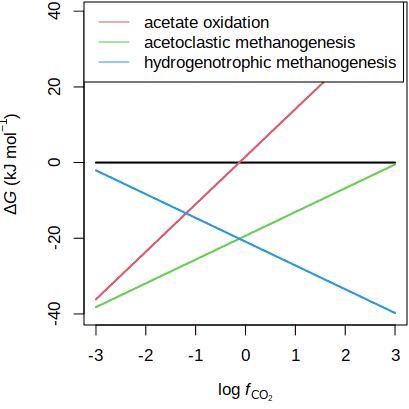Gibbs energies of acetate oxidation and methanogenesis (after Mayumi et al., 2013).

``````Adat <- lapply(c(-3, 3), function(logfCO2) {
basis("CO2", logfCO2)
data.frame(logfCO2,
rxnfun(c(0, 0))\$A,
rxnfun(c(-1, 0))\$A,
rxnfun(c(-1, 1))\$A,
rxnfun(c(0, 1))\$A
)
})
matplot(Adat[, 1], -Adat[, -1]/1000, type = "l", lty = 1, lwd = 2,
xlab = axis.label("CO2"), ylab = axis.label("DG", prefix = "k"))
legend("topleft", c("acetate oxidation", "acetoclastic methanogenesis",
"hydrogenotrophic methanogenesis"), lty = 1, col = 2:4)``````

Let’s not forget to clear the system settings, which were modified by `basis()`, before running other calculations:

``reset()``

# Using `affinity()`

`affinity()` offers calculations of chemical affinity of formation reactions over a configurable range of T, P, and activities of basis species.

## From affinity to diagrams

By formation reaction is meant the stoichiometric requirements for formation of one mole of any species from the basis species. The `species()` function is used to set these formed species. Let’s consider the stoichiometry of some aqueous sulfur-bearing species. Here we use `basis()` with a keyword to load a preset basis definition. To use Eh as a variable, the electron (e-) should be in the basis, so we use the keyword (`basis("CHNOSe")`). Some available keywords are `CHNOS` (including CO2, H2O, NH3, H2S, and O2), `CHNOS+` (also including H+), and `CHNOSe` (including H+, and e- instead of O2). See `?basis` for more options. What is `SO42-`? Is it 1 S, 4 O, and 2 negative charges, or 1 S, 42 O, and 1 negative charge? The ambiguity of a digit that could belong to the coefficient for the following charge or to that for the preceding element is why formulas in CHNOSZ are written with the number of charges after the + or - symbol. `SO4-2` is unambiguously parsed as 1 S, 4 O and 2 negative charges.

``basis("CHNOSe")``
``species(c("H2S", "HS-", "HSO4-", "SO4-2"))``
``````##   CO2 H2O NH3 H2S e-  H+ ispecies logact state  name
## 1   0   0   0   1  0   0       65     -3    aq   H2S
## 2   0   0   0   1  0  -1       22     -3    aq   HS-
## 3   0   4   0   1 -8  -9       25     -3    aq HSO4-
## 4   0   4   0   1 -8 -10       24     -3    aq SO4-2``````

Aqueous species are assigned default activities of 10-3 (`logact` is -3). Now, we can use `affinity()` to calculate the affinities of the formation reactions of each of the species. R’s `unlist()` is used here to turn the list of values of affinity into a numeric object that can be printed in a couple of lines (note that the names correspond to `ispecies` above):

``unlist(affinity()\$values)``
```## affinity: temperature is 25 ºC
```
```## affinity: pressure is Psat
```
```## subcrt: 10 species at 25 ºC and 1 bar (wet) [energy units: J]
```
``````##       65       22       25       24
## -4.00000 -3.98775 76.30176 81.32264``````

The values returned by `affinity()` are dimensionless, i.e. A/(2.303RT). Although `subcrt()` can also calculate affinities (in units of cal/mol or J/mol), the main advantage of `affinity()` is that it can perform calculations on an arbitrary grid of T, P, or activities of basis species. This is the foundation for making many types of diagrams that are useful in geochemistry.

Given the values of affinity, the `diagram()` function identifies the species that has the maximum affinity at each grid point. The result is equivalent to a “predominance diagram” (Dick, 2019Dick JM. 2019. CHNOSZ: Thermodynamic calculations and diagrams for geochemistry. Frontiers in Earth Science 7: 180. doi: 10.3389/feart.2019.00180), where the fields represent the predominant aqueous species and the boundaries are equal-activity lines. If both aqueous species and minerals are present, it is common practice to assign a constant activity to all aqueous species and unit activity (i.e. log activity = 0) for minerals. More sophisticated diagrams can be made by showing the solubility contours of a metal on the diagram; see `demo(contour)` for an example.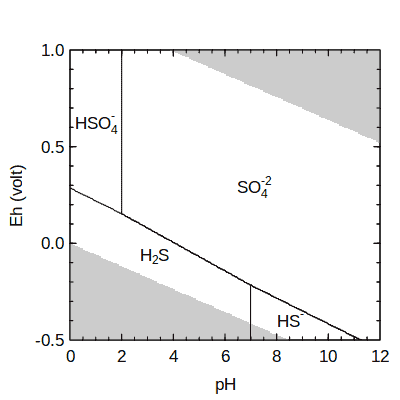Aqueous sulfur species at 25 °C.

Let’s use `diagram()` to make a simple Eh-pH diagram for aqueous species in the system S-O-H. After running the `basis()` and `species()` commands above, we can calculate the affinities on an Eh-pH grid. With the default settings in `diagram()`, areas beyond the stability limits of water are colored gray. This can be changed by setting the `limit.water` argument to FALSE (to not show the stability region of water) or TRUE (to plot the main diagram only in the stable region of water). The names of species that can be parsed as chemical formulas are formatted with subscripts and superscripts; if this is not desired, set `format.names = FALSE`.

``````a <- affinity(pH = c(0, 12), Eh = c(-0.5, 1))
diagram(a, limit.water = TRUE)``````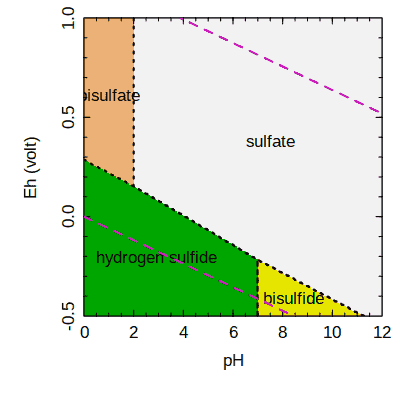The same plot, with different colors and labels.

Other arguments in `diagram()` can be used to control the line type (`lty`), width (`lwd`), and color (`col`), field colors (`fill`), and species labels (`name`). Additional arguments are passed to R’s plotting functions; here, we use `las` to change the orientation of axes labels. Another function, `water.lines()`, can be used to draw lines at the water stability limits:

``````diagram(a, fill = "terrain", lwd = 2, lty = 3,
names = c("hydrogen sulfide", "bisulfide", "bisulfate", "sulfate"),
las = 0)
water.lines(a, col = 6, lwd = 2)``````

See the demos in the package for other examples of Eh-pH diagrams. In particular, `demo(Pourbaix)` shows how to plot the concentrations of metals as equisolubility (or isosolubility) lines on Eh-pH diagrams (Pourbaix, 1974Pourbaix M. 1974. Atlas of Electrochemical Equilibria in Aqueous Solutions. 2nd ed. Houston, Texas; Brussels: National Association of Corrosion Engineers; CEBELCOR. Available at https://www.worldcat.org/oclc/563921897. ).

Mineral stability diagrams often depict activity ratios, e.g. log (aCa+2/aH+2), on one or both axes. The variables used for potential calculations in CHNOSZ include only a single chemical activity, e.g. log aCa+2. However, you can set pH = 0 to generate diagrams that are geometrically equivalent to those calculated using activity ratios, and use `ratlab()` to make the axes labels for the ratios. Moreover, `diagram()` has a “saturation” option that can be used to draw saturation limits for minerals that do not contain the conserved basis species. See `demo(saturation)` for an example that uses activity ratios on the axes and plots saturation limits for calcite, magnesite, dolomite, and brucite on a diagram for the H2O–CO2–CaO–MgO–SiO2 system. That demo can be used as a template to produce a wide range of diagrams similar to those in Bowers et al. (1984Bowers TS, Jackson KJ, Helgeson HC. 1984. Equilibrium Activity Diagrams for Coexisting Minerals and Aqueous Solutions at Pressures and Temperatures to 5 Kb and 600°C. Heidelberg: Springer-Verlag. doi: 10.1007/978-3-642-46511-6).

## Retrieving species in a chemical system

Most of the diagrams in this vignette are made by giving the names of all the species. However, it can be tedious to find all the species by manually searching the OBIGT database. The `retrieve()` function can be used to identify the available species that contain particular chemical elements. For example, to get Mn-bearing aqueous species and minerals including the single element, oxides and oxyhydroxides, use this:

``retrieve("Mn", c("O", "H"), "aq")``
``````##  MnO4-   Mn+2   Mn+3 MnO4-2  MnOH+    MnO HMnO2- MnO2-2
##    438    447    524    525    617    618    619    620``````
``retrieve("Mn", c("O", "H"), "cr")``
``````##     MnO    MnO2   Mn2O3   Mn3O4      Mn Mn(OH)2
##    2044    2045    2046    2047    2048    2049``````

Below, these commands are used to identify the species in an Eh-pH diagram for the Mn-O-H system at 100 °C. This diagram includes Mn oxides (pyrolusite, bixbyite, hausmannite), Mn(OH)2, and aqueous Mn species.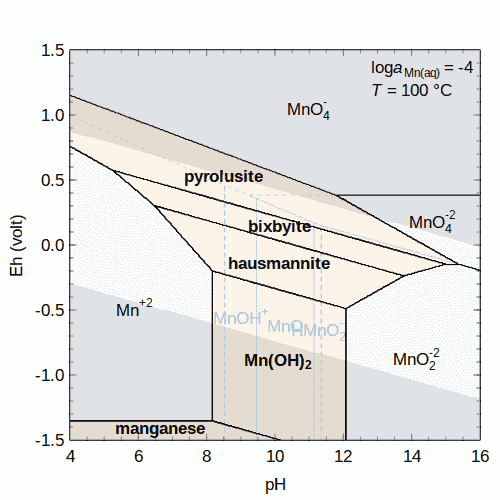Eh-pH diagram for the Mn-O-H system.

``````# Set decimal logarithm of activity of aqueous species,
# temperature and plot resolution
logact <- -4
T <- 100
res <- 400
basis(c("Mn+2", "H2O", "H+", "e-"))
iaq <- retrieve("Mn", c("O", "H"), "aq")
species(iaq, logact)
aaq <- affinity(pH = c(4, 16, res), Eh = c(-1.5, 1.5, res), T = T)
# Show names for only the metastable species here
names <- names(iaq)
names[!names(iaq) %in% c("MnOH+", "MnO", "HMnO2-")] <- ""
diagram(aaq, lty = 2, col = "#4169E188", names = names, col.names = 4)
# Overlay mineral stability fields
icr <- retrieve("Mn", c("O", "H"), "cr")
# Supply the previous result from affinity() to use
# argument recall (for plotted variables and T)
acr <- affinity(aaq)
diagram(acr, add = TRUE, bold = acr\$species\$state=="cr", limit.water = FALSE)
legend <- c(
bquote(log * italic(a)["Mn(aq)"] == .(logact)),
bquote(italic(T) == .(T) ~ degree*C)
)
legend("topright", legend = as.expression(legend), bty = "n")``````

This introductory vignette shows examples for diagrams with a single metal. Other functions are available to make diagrams for multiple metals; see the vignette Diagrams with multiple metals for more information.

## Mosaic diagrams

If sulfur is an element in the basis species, then we should consider that its speciation is sensitive to Eh and pH, as shown in a previous diagram. Mosaic diagrams account for speciation of the basis species and are useful for visualizing the stabilities of many types of minerals including oxides, sulfides, and carbonates. These diagrams are made by constructing individual diagrams for the possible basis species. The individual diagrams are then combined, each one contributing to the final diagram only in the range of stability of the corresponding basis species.

Let’s use `mosaic()` to make a diagram for aqueous species and minerals in the Cu-S-Cl-H2O system. To know what aqueous copper chloride complexes are available in the database, we can use a fuzzy search:

``info(" CuCl")``
```## info.approx: ' CuCl' is ambiguous; has approximate matches to 7 species:
```
```##  "CuCl3-2" "CuCl+"   "CuCl2"   "CuCl3-"  "CuCl4-2" "CuCl"    "CuCl2-"
```

Next we define the basis, and set the activities of the H2S and Cl- basis species. These represent the total activity of S and Cl in the system, which are distributed among the minerals and aqueous species. Aqueous copper chloride complexes and four minerals are added. Be sure to include `add = TRUE` in the second call to `species()`; otherwise, the minerals would simply replace the previously added aqueous species.

``````basis(c("Cu", "H2S", "Cl-", "H2O", "H+", "e-"))
basis("H2S", -6)
basis("Cl-", -0.7)
species(c("CuCl", "CuCl2-", "CuCl3-2", "CuCl+", "CuCl2", "CuCl3-", "CuCl4-2"))
species(c("chalcocite", "tenorite", "cuprite", "copper"), add = TRUE)``````

Note that chalcocite (Cu2S) undergoes phase transitions. To get the temperatures of the phase transitions from `thermo()\$OBIGT` (in Kelvin, regardless of the `T.units()`), we can use `info()`. We see that at 200 °C (473.15 K) the second phase is stable; this one is automatially used by CHNOSZ for this diagram.

``info(info("chalcocite", c("cr", "cr2", "cr3")))\$T``
``##   376  717 1400``

We use `mosaic()` to generate and combine diagrams for each candidate basis species (H2S, HS-, HSO4-, or SO4-2) as a function of Eh and pH. The key argument is `bases`, which identifies the candidate basis species, starting with the one in the current basis. The other arguments, like those of `affinity()`, specify the ranges of the variables; `res` indicates the grid resolution to use for each variable (the default is 256). The first call to `diagram()` plots the species of interest; the second adds the predominance fields of the basis species. We also use `water.lines()` to draw dashed blue lines at the water stability limits: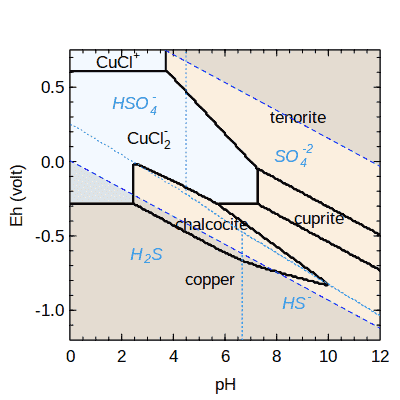Copper minerals and aqueous complexes with chloride, 200 °C.

``````T <- 200
res <- 200
bases <- c("H2S", "HS-", "HSO4-", "SO4-2")
m1 <- mosaic(bases, pH = c(0, 12, res), Eh=c(-1.2, 0.75, res), T=T)
diagram(m1\$A.species, lwd = 2)
diagram(m1\$A.bases, add = TRUE, col = 4, col.names = 4, lty = 3,
italic = TRUE)
water.lines(m1\$A.species, col = "blue1")``````

The diagrams are combined according to the relative abundances of the different possible basis species listed in `bases` along with a term for the Gibbs energy of mixing (see `?mosaic`). The smooth transitions between basis species can result in curved field boundaries, in this case around the chalcocite field. If we added the argument `blend = FALSE`, the diagrams would instead be assembled using the single predominant basis species at any point on the Eh-pH grid, and all of the line segments would be straight.

The reactions used to make this diagram are balanced on Cu, so that no Cu appears in reactions between any two other species (minerals or aqueous species). If `diagram()` is run with `balance = 1`, then the reactions are balanced on formula units. That is, one mole of the mineral formulas appears on each side of the reaction, with the possibility of Cu appearing as an additional species to conserve the elements. This may be problematic, as Cu would be be present in some reactions in Eh-pH space where it is not a stable phase. However, it is common in low-temperature aqueous geochemical calculations to “turn off” particular redox reactions that are not thought to attain equilibrium, so decoupling a species from equilibrium may be justified in some circumstances. Changing the balance to 1 results in the loss of the tenorite stability field and extension of chalcocite stability to lower pH, as shown in Figure 5a of Caporuscio et al. (2017Caporuscio FA, Palaich SEM, Cheshire MC, Jové Colón CF. 2017. Corrosion of copper and authigenic sulfide mineral growth in hydrothermal bentonite experiments. Journal of Nuclear Materials 485: 137–146. doi: 10.1016/j.jnucmat.2016.12.036).

## T, P, activity transects

Above, we used evenly-spaced grids of chemical activities of basis species; the ranges of variables were given by two or three values (minimum, maximum, and optionally resolution). `affinity()` can also perform calculations along a transect, i.e. a particular path along one or more variables. A transect is calculated when there are four or more values assigned to the variable(s). Let’s use this feature to calculate affinities (negative Gibbs energies) of methanogenesis and biosynthetic reactions in a hydrothermal system.

Some results of mixing calculations for seawater and vent fluid from the Rainbow hydrothermal field, calculated using EQ3/6 by Shock and Canovas (2010Shock EL, Canovas P. 2010. The potential for abiotic organic synthesis and biosynthesis at seafloor hydrothermal systems. Geofluids 10(1-2): 161–192. doi: 10.1111/j.1468-8123.2010.00277.x), are included in a data file in CHNOSZ. Reading the file with R’s `read.csv()`, we set `check.names = FALSE` to preserve the `NH4+` column name (which is not a syntactically valid variable name):

``````file <- system.file("extdata/cpetc/SC10_Rainbow.csv", package = "CHNOSZ")
rb <- read.csv(file, check.names = FALSE)``````

We take a selection of the species from Shock and Canovas (2010) with activities equal to 10-6; aqueous CH4 is assigned an activity of 10-3. We will write the synthesis reactions of organic species in terms of these basis species: The constant activity of CH4 is a simplification of the calculation reported by Shock and Canovas (2010). The code here could be expanded to vary the activity of CH4.

``````basis(c("CO2", "H2", "NH4+", "H2O", "H2S", "H+"))
species("CH4", -3)
"CH4", "leucine", "tryptophan", "n-nonanoic acid"), -6)``````

Now we can calculate affinities along the transect of changing temperature and activities of five basis species. Each variable is given as a named argument; `NH4+` must be quoted. A shorter expression would use R’s `do.call()` to construct the function call: `do.call(``affinity``, as.list(rb))`. The target of the conversion is `G`, or free energy, from `logK`. That conversion requires temperature in Kelvin, which is obtained by conversion from °C. We finish with a negation (affinity is negative Gibbs energy) and scaling from cal to kcal. Using R’s `lapply()` to run `convert()` for each species, we first convert the affinity from dimensionless values (A/(2.303RT)) to J/mol. A second call to `convert()` is used to obtain energies in cal/mol, and these are finally converted to kcal/mol.

``````a <- affinity(T = rb\$T, CO2 = rb\$CO2, H2 = rb\$H2,
`NH4+` = rb\$`NH4+`, H2S = rb\$H2S, pH = rb\$pH)
T <- convert(a\$vals[], "K")
a\$values <- lapply(a\$values, convert, "G", T)
a\$values <- lapply(a\$values, convert, "cal")
a\$values <- lapply(a\$values, `*`, -0.001)``````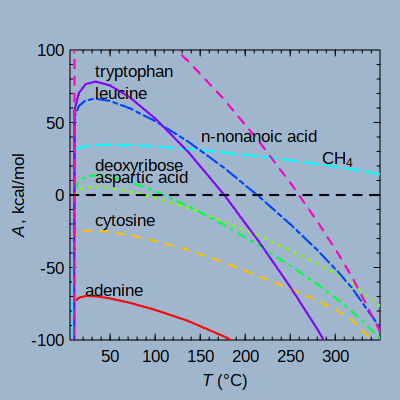Affinities of organic synthesis in a hydrothermal system, after Shock and Canovas (2010).

Finally, we use `diagram()` to plot the results. Although only temperature is shown on the x axis, pH and the activities of CO2, H2, NH4+, and H2S are also varied according to the data in `rb`. By default, `diagram()` attempts to scale the affinities by dividing by the reaction coefficients of a shared basis species (in this case, CO2). To override that behavior, we set `balance = 1` to plot the affinities of the formation reactions as written (per mole of the product species).

``````diagram(a, balance = 1, ylim = c(-100, 100), ylab = quote(italic(A)*", kcal/mol"),
col = rainbow(8), lwd = 2, bg = "slategray3")
abline(h = 0, lty = 2, lwd = 2)``````

When making line plots, `diagram()` automatically places the labels near the lines. The additional arguments `adj` and `dy` can be used to fine-tune the positions of the labels (they are used in a couple of examples below). If labeling of the lines is not desired, add e.g. `legend.x = "topright"` to make a legend instead, or `names = FALSE` to prevent any plotting of the names.

## Buffers

There is one other feature of `affinity()` that can be mentioned here. Can we go the other direction: calculate the activities of basis species from the activities of the species of interest? This question relates to the concept of chemical activity buffers. In CHNOSZ there are two ways to perform buffer calculations:

1. Assign the name of a buffer (listed in `thermo()\$buffer`) to the basis species:
• more versatile (multiple activities can be buffered, e.g. both S2 and O2 by pyrite-pyrrhotite-magnetite);
• the buffers are active in calculations of affinity of other species;
• use `mod.buffer()` to change or add buffers in `thermo()\$buffer`;
• `demo(buffer)` uses it for mineral buffers (solid lines).
1. Use the `type` argument of `diagram()` to solve for the activity of the indicated basis species:
• more convenient (the buffers come from the currently defined species of interest), but only a single basis species can be buffered, and it’s not used in the calculation of affinity;
• `demo(buffer)` uses it for aqueous organic species as buffers (dotted and dashed lines).

As an example of method 1, let’s look at the pyrite-pyrrhotite-magnetite (PPM) buffer at 300 °C. For other examples, see `?buffer` and `demo(protbuff)` (hypothetical buffer made of proteins). Without the buffer, the basis species have default activities of zero. Under these conditions, the minerals are not in equilibrium, as shown by their different affinities of formation:

``````basis(c("FeS2", "H2S", "O2", "H2O"))
species(c("pyrite", "magnetite"))
species("pyrrhotite", "cr2")``````

The affinity of formation of pyrite happens to be zero because it is identical to one of the selected basis species.

``unlist(affinity(T = 300, P = 100)\$values)``
``````##     1956
## -20.6134``````

We use `mod.buffer()` to choose the `cr2` phase of pyrrhotite, which is stable at this temperature (see above for how to get this information for minerals with phase transitions). Then, we set up H2S and O2 to be buffered by PPM, and inspect their buffered activities:

``mod.buffer("PPM", "pyrrhotite", "cr2")``
```## mod.buffer: changed state and/or logact of pyrrhotite in PPM buffer
```
``basis(c("H2S", "O2"), c("PPM", "PPM"))``
``unlist(affinity(T = 300, P = 100, return.buffer = TRUE)[1:3])``
``````##       H2S        O2      FeS2
##  -2.36691 -36.49303   0.00000``````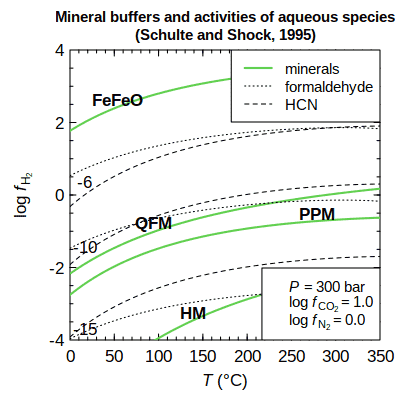Values of logfH2 corresponding to mineral buffers or to given activities of aqueous species.

Et voilà! We have found logaH2S and logfO2 that are compatible with the coexistence of the three minerals. Under these conditions, the affinities of formation reactions of the minerals in the buffer are all equal to zero:

``````unlist(affinity(T = 300, P = 100)\$values)
## 2031 1999 2036
##    0    0    0``````

Another example, based on Figure 6 of Schulte and Shock (1995Schulte MD, Shock EL. 1995. Thermodynamics of Strecker synthesis in hydrothermal systems. Origins of Life and Evolution of the Biosphere 25(1-3): 161–173. doi: 10.1007/BF01581580), is given in `demo(buffer)`. Here, values of logfH2 buffered by minerals or set by equilibrium with given activities of aqueous species are calculated using the two methods:

``demo(buffer)``

# Equilibrium

Above we considered this question: for equal activities of species, what are the affinities of their formation reactions from basis species? Turning the question around, we would like to know: for equal affinities of formation reactions, what are the activities of species? The first question is about non-equilibrium conditions; the second is about equilibrium.

Before presenting some examples, it is helpful to know about the limitations of the functions. CHNOSZ does not take account of all possible reactions in the speciation of a system. Instead, it assumes that the total activity of species in the system is set by the activity of one basis species. When activity coefficients are assumed to be zero, activities are equal to concentration and we can refer to “total activity”. If the ionic strength is specified, `nonideal()` (see below) can be used to calculate activity coefficients. This balanced, or conserved, basis species must be present (with a positive or negative coefficient) in the formation reactions of all species considered.

For a given total activity of the balanced basis species, activities of the species can be found such that the affinities of the formation reactions are all equal. This is an example of metastable equilibrium. With additional constraints, the affinities of the formation reactions are not only equal to each other, but equal to zero. This is total equilibrium. An example of total equilibrium was given above for the PPM buffer. In contrast, models for systems of organic and biomolecules often involve metastable equilibrium constraints.

## Equilibration methods

The `equilibrate()` function in CHNOSZ automatically chooses between two methods for calculating equilibrium. The method based on the Boltzmann equation is fast, but is applicable only to systems where the coefficient on the balanced basis species in each of the formation reactions is one. The reaction-matrix method is slower, but can be applied to systems were the balanced basis species has reaction coefficients other than one.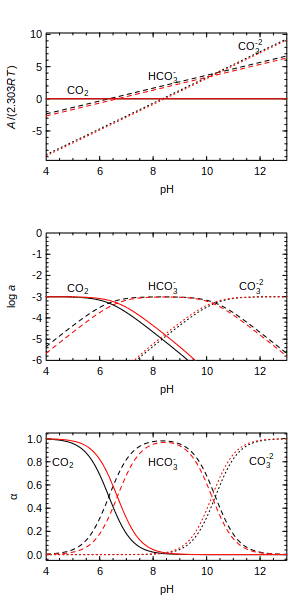Three views of carbonate speciation: affinity, activity, degree of formation.

The distribution of aqueous carbonate species as a function of pH (a type of Bjerrum plot) is a classic example of an equilibrium calculation. We can begin by plotting the affinities of formation, for equal activities of the species, calculated at 25 °C and 150 °C. Here, CO2 is in the basis, so it has zero affinity, which is greater than the affinities of HCO3- and CO3-2 at low pH. To avoid overplotting the lines, we offset the species labels in the y direction using the `dy` argument:

``````par(mfrow = c(3, 1))
basis("CHNOS+")
species(c("CO2", "HCO3-", "CO3-2"))
a25 <- affinity(pH = c(4, 13))
a150 <- affinity(pH = c(4, 13), T = 150)
diagram(a25, dy = 0.4)
diagram(a150, add = TRUE, names = FALSE, col = "red")``````

Now we use `equilibrate()` to calculate the activities of species. Our balancing constraint is that the total activity of C is 10-3. This shows a hypothetical metastable equilibrium; we know that for true equilibrium the total activity of C is affected by pH.

``````e25 <- equilibrate(a25, loga.balance = -3)
e150 <- equilibrate(a150, loga.balance = -3)
diagram(e25, ylim = c(-6, 0), dy = 0.15)
diagram(e150, add = TRUE, names = FALSE, col = "red")``````

To display the species distribution, or degree of formation, add `alpha = TRUE` to the argument list:

``````diagram(e25, alpha = TRUE, dy = -0.25)
diagram(e150, alpha = TRUE, add = TRUE, names = FALSE, col = "red")``````

## Complete equilibrium: Solubility

It is important to remember that `equilibrate()` calculates an equilibrium distribution of species for a given total activity of the conserved basis species. For instance, the previous diagram shows the relative abundances of CO2, HCO3-, and CO3-2 as a function of pH assuming that the possible reactions between species are all balanced on 1 C and the total activity of C is constant. Although this assumption of metastable equilibrium is useful for making many types of diagrams for aqueous species, the aqueous solutions would not be in equilibrium with other phases, including gases or solids such as carbon dioxide or calcite.

Moving away from metastable equilibrium to complete equilibrium actually involves a simplification of the computations. Instead of finding the activities of aqueous species where the affinities of formation reactions are equal to each other, equilibrium occurs when the affinities of all formation reactions are equal to zero. However, the solubility calculation comes with a different constraint: all reactions between the pure substance that is being dissolved and any of the possible formed aqueous species must be able to be balanced on the same element (usually the main metal in the system). This balancing constraint can be expressed as a conserved basis species, i.e. one that is present in non-zero quantity in the formation reactions of all species considered.

The `solubility()` function provides a way to compute activities of aqueous species in equilibrium with one or more minerals or gases. The following example for corundum (Al2O3) is based on Figure 15 of Manning (2013Manning CE. 2013. Thermodynamic modeling of fluid-rock interaction at mid-crustal to upper-mantle conditions. Reviews in Mineralogy and Geochemistry 76(1): 135–164. doi: 10.2138/rmg.2013.76.5). To more closely reproduce the diagram, we use superseded thermodynamic data that are kept in the `SLOP98` optional data file.

The basis species are defined with the main element (Al) first. The mineral we want to dissolve, corundum, is loaded as a formed species. The aqueous species that can form by dissolution of corundum are listed in the `iaq` argument of `solubility()`. The next arguments describe the variables for the `affinity()` calculations. Note that setting `IS` to 0 has no effect on the calculations, but signals `diagram()` to label the y axis with logarithm of molality instead of logarithm of activity. An additional argument, `in.terms.of`, is used to compute the total molality of Al in solution, that is, twice the number of moles of Al2O3 that are dissolved.

`diagram()` is used twice, first to plot the total molality of Al, then the concentrations of the individual species, using `adj` and `dy` to adjust the positions of labels in the x- and y-directions. At the end of the calculation, we use `reset()` to restore the default thermodynamic database.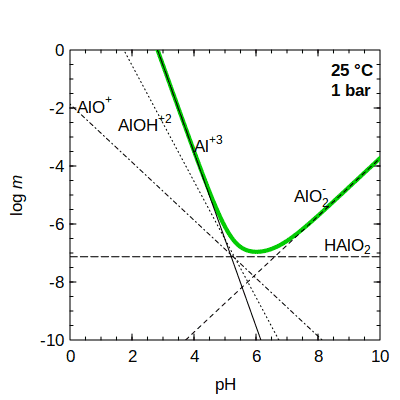Solubility of corundum (green line) and equilibrium concentrations of aqueous species (black lines).

``````add.OBIGT("SLOP98")
basis(c("Al+3", "H2O", "H+", "O2"))
species("corundum")
iaq <- c("Al+3", "AlO2-", "AlOH+2", "AlO+", "HAlO2")
s <- solubility(iaq, pH = c(0, 10), IS = 0, in.terms.of = "Al+3")
diagram(s, type = "loga.balance", ylim = c(-10, 0), lwd = 4, col = "green3")
diagram(s, add = TRUE, adj = c(0, 1, 2.1, -0.2, -1.5), dy = c(0, 0, 4, -0.3, 0.1))
legend("topright", c("25 °C", "1 bar"), text.font = 2, bty = "n")``````
``reset()``

Other examples of using `solubility()` are available in CHNOSZ. See `demo(solubility)` for calculations of the solubility of CO2(gas) and calcite as a function of pH and temperature. The calculation considers the stoichiometric dissolution of calcite, in which CaCO3 dissociates to form equal quantities of Ca+2 and CO3-2 ions. Adding in activity coefficients, an example in `?solubility` uses the `find.IS` option to find the final ionic strength for dissolving calcite into pure water. `demo(gold)` shows calculations of the solubility of gold as a function of pH and T as well as oxygen fugacity set by diferent mineral buffers, and considers ionic strength effects on activity coefficients, so that activities are transformed to molalities (see below).

# Activity coefficients

For calculating activity coefficients of charged species, `nonideal()` uses the extended Debye–Hückel equation as parameterized by Helgeson et al. (1981Helgeson HC, Kirkham DH, Flowers GC. 1981. Theoretical prediction of the thermodynamic behavior of aqueous electrolytes at high pressures and temperatures: IV. Calculation of activity coefficients, osmotic coefficients, and apparent molal and standard and relative partial molal properties to 600°C and 5 Kb. American Journal of Science 281(10): 1249–1516. doi: 10.2475/ajs.281.10.1249) for NaCl-dominated solutions to high pressure and temperature, or optionally using parameters described in Chapter 3 of Alberty (2003Alberty RA. 2003. Thermodynamics of Biochemical Reactions. Hoboken, New Jersey: Wiley-Interscience. Available at https://www.worldcat.org/oclc/51242181. ), which are applicable to relatively low-temperature biochemical reactions. The activity coefficients are calculated as a function of ionic strength (I), temperature, and charge of each species, without any other species-specific parameters. Using the default `Helgeson` method, the extended term parameter (“B-dot”) is derived from data of Helgeson (1969Helgeson HC. 1969. Thermodynamics of hydrothermal systems at elevated temperatures and pressures. American Journal of Science 267(7): 729–804. doi: 10.2475/ajs.267.7.729) and Helgeson et al. (1981), and extrapolations of Manning et al. (2013Manning CE, Shock EL, Sverjensky DA. 2013. The chemistry of carbon in aqueous fluids at crustal and upper-mantle conditions: Experimental and theoretical constraints. Reviews in Mineralogy and Geochemistry 75(1): 109–148. doi: 10.2138/rmg.2013.75.5).

## From activity to molality

Following the main workflow of CHNOSZ, `nonideal()` normally does not need to be used directly. Intead, invoke the calculations by setting the `IS` argument in `subcrt()` or `affinity()`. There are a few things to remember when using activity coefficients:

• H+ is assumed to behave ideally, so its activity coefficient is 1 for any ionic strength. You can calculate activity coefficients of H+ by running `thermo("opt\$ideal.H" = FALSE)`.

• Using `subcrt()` with `IS` not equal to zero, calculated values of `G` are the adjusted Gibbs energy at specified ionic strength (denoted by ΔG°(I); Alberty, 1996Alberty RA. 1996. Recommendations for nomenclature and tables in biochemical thermodynamics. European Journal of Biochemistry 240(1): 1–14. doi: 10.1111/j.1432-1033.1996.0001h.x).

• Using `subcrt()` with `IS` not equal to zero, values in the `logact` argument stand for log molality of aqueous species in affinity calculations.

• Using `affinity()` with `IS` not equal to zero, the following values stand for log molality of aqueous species:

• values of `logact` set by `basis()`;
• values of `logact` set by `species()`.
• Using `equilibrate()` on the output of affinity calculated with `IS` not equal to zero, the following values stand for log molality of aqueous species:

• the value of `loga.balance` used by `equilibrate()` (i.e., logarithm of total molality of the balancing basis species);
• values of `loga.equil` returned by `equilibrate()`.

In other words, the activation of activity coefficients effects a transformation from activity to molality in the main workflow. A simple but comprehensive series of calculations demonstrating these tranformations is in `inst/tinytest/test-logmolality.R`.

Because it is not possible to dynamically change the names of arguments in the functions, the user should be aware of the transformations mentioned above. However, the labels on diagrams can be automatically adjusted; accordingly, activities of aqueous species are relabeled as molalities by `diagram()` when `IS` is used in the calculation of `affinity()`.

## Biochemical example

For the following calculations, we change the nonideality method to `Alberty`; this is a simpler formulation with parameters that are suitable for biochemical species at relatively low temperatures:

``oldnon <- nonideal("Alberty")``
```## nonideal: setting nonideal option to use Alberty equation
```

Let’s take a look at calculated activity coefficients at two temperatures and their effect on the standard Gibbs energies of formation (ΔG°f) of species with different charge:

``````subcrt(c("MgATP-2", "MgHATP-", "MgH2ATP"),
T = c(25, 100), IS = c(0, 0.25), property = "G")\$out``````
```## subcrt: 3 species at 2 values of T (ºC) and P (bar) (wet) [energy units: J]
```
```## nonideal: calculations for MgC10H12N5O13P3-2, MgC10H13N5O13P3- (Alberty equation)
```
```## nonideal: calculations for MgC10H14N5O13P3 (Setchenow equation)
```
``````## \$`MgATP-2`
##     T       P        G    loggam   IS
## 1  25 1.00000 -3236780  0.000000 0.00
## 2 100 1.01322 -3265305 -0.662587 0.25
##
## \$`MgHATP-`
##     T       P        G    loggam   IS
## 1  25 1.00000 -3267679  0.000000 0.00
## 2 100 1.01322 -3303769 -0.165647 0.25
##
## \$MgH2ATP
##     T       P        G      loggam   IS
## 1  25 1.00000 -3289461  0.00000000 0.00
## 2 100 1.01322 -3326343 -0.00195159 0.25``````

The logarithms of the activity coefficients (`loggam`) are more negative for the higher-charged species, as well as at higher temperature, and have a stabilizing effect. That is, the adjusted Gibbs energies at I > 0 are less than the standard Gibbs energies at I = 0.

We can use these calculations to make some speciation plots, similar to Figures 1.2–1.5 in Alberty (2003). These figures show the distribution of differently charged species of adenosine triphosphate (ATP) as a function of pH, and the average number of H+ and Mg+2 bound to ATP in solution as a function of pH or pMg (-logaMg+2).

Use `info()` to see what ATP species are available. The sources of high-temperature thermodynamic data for these species are two papers by LaRowe and Helgeson (2006a; LaRowe DE, Helgeson HC. 2006a. Biomolecules in hydrothermal systems: Calculation of the standard molal thermodynamic properties of nucleic-acid bases, nucleosides, and nucleotides at elevated temperatures and pressures. Geochimica et Cosmochimica Acta 70(18): 4680–4724. doi: 10.1016/j.gca.2006.04.0102006b)LaRowe DE, Helgeson HC. 2006b. The energetics of metabolism in hydrothermal systems: Calculation of the standard molal thermodynamic properties of magnesium-complexed adenosine nucleotides and NAD and NADP at elevated temperatures and pressures. Thermochimica Acta 448(2): 82–106. doi: 10.1016/j.tca.2006.06.008.

``info(" ATP")``
```## info.approx: ' ATP' is ambiguous; has approximate matches to 14 species:
```
```##   "ATP-4"    "HATP-3"   "H2ATP-2"  "H3ATP-"   "H4ATP"    "dATP-4"
##   "dHATP-3"  "dH2ATP-2" "dH3ATP-"  "dH4ATP"   "MgATP-2"  "MgHATP-"
##  "MgH2ATP"  "Mg2ATP"
```

The plots for this system in Alberty’s book were made for I = 0.25 M and T = 25 °C. As a demonstration of CHNOSZ’s capabilities, we can assign a temperature of 100 °C.

``T <- 100``

Use the following commands to set the basis species, add the variously protonated ATP species, calculate the affinities of the formation reactions, equilibrate the system, and make a degree of formation (α) or mole fraction diagram. This is similar to Figure 1.3 of Alberty (2003), but is calculated for I = 0 M and T = 100 °C: To make the code more readable, commands for plotting titles and legends are not shown. All of the commands are available in the source of this document.

``````basis("MgCHNOPS+")
species(c("ATP-4", "HATP-3", "H2ATP-2", "H3ATP-", "H4ATP"))
a <- affinity(pH = c(3, 9), T = T)
e <- equilibrate(a)
d <- diagram(e, alpha = TRUE, tplot = FALSE)``````

Note that we have saved the numeric results of `diagram()`, i.e. the degrees of formation of the species (α). With that, we can calculate and plot the average number of protons bound per ATP molecule. To do so, we use R’s `rbind()` and `do.call()` to turn `alpha` into a matrix, then multiply by the number of protons bound to each species, and sum the columns to get the total (i.e. average proton number, NH+):

``````alphas <- do.call(rbind, d\$plotvals)
nH <- alphas * 0:4
Hlab <- substitute(italic(N)[H^`+`])
plot(a\$vals[], colSums(nH), type = "l", xlab = "pH", ylab=Hlab, lty=2, col=2)``````

Adding the `IS` argument to `affinity()`, we can now plot NH+ at the given ionic strength. Here we set `plot.it = FALSE` in `diagram()` because we use the computed α to make our own plot. This is similar to Figure 1.3 of Alberty (2003), but at higher temperature:

``````a <- affinity(pH = c(3, 9), IS = 0.25, T = T)
e <- equilibrate(a)
d <- diagram(e, alpha = TRUE, plot.it = FALSE)
alphas <- do.call(rbind, d\$plotvals)
nH <- alphas * 0:4
lines(a\$vals[], colSums(nH))``````

Next, we add the Mg+2-complexed ATP species:

``species(c("MgATP-2", "MgHATP-", "MgH2ATP", "Mg2ATP"), add = TRUE)``

Here is a function to calculate and plot NH+ for a given pMg:

``````Hplot <- function(pMg, IS = 0.25) {
basis("Mg+2", -pMg)
a <- affinity(pH = c(3, 9), IS = IS, T = T)
e <- equilibrate(a)
d <- diagram(e, alpha = TRUE, plot.it = FALSE)
alphas <- do.call(rbind, d\$plotvals)
NH <- alphas * c(0:4, 0, 1, 2, 0)
lines(a\$vals[], colSums(NH), lty = 7 - pMg, col = 7 - pMg)
}``````

With that function in hand, we plot the lines corresponding to pMg = 2 to 6. This is similar to Figure 1.4 of Alberty (2003):

``````plot(c(3, 9), c(0, 2), type = "n", xlab = "pH", ylab = Hlab)
lapply(2:6, Hplot)``````

The next function calculates and plots the average number of Mg+2 bound to ATP (NMg+2) for a given pH. Here we multiply `alpha` by the number of Mg+2 in each species, and negate logaMg+2 (the variable used in `affinity()`) to get pMg:

``````Mgplot <- function(pH, IS = 0.25) {
basis("pH", pH)
a <- affinity(`Mg+2` = c(-2, -7), IS = IS, T = T)
e <- equilibrate(a)
d <- diagram(e, alpha = TRUE, plot.it = FALSE)
alphas <- do.call(rbind, d\$plotvals)
NMg <- alphas * species()\$`Mg+`
lines(-a\$vals[], colSums(NMg), lty = 10 - pH, col = 10 - pH)
}``````

Using that function, we plot the lines corresponding to pH = 3 to 9. This is similar to Figure 1.5 of Alberty (2003):

``````Mglab <- substitute(italic(N)[Mg^`+2`])
plot(c(2, 7), c(0, 1.2), type = "n", xlab = "pMg", ylab = Mglab)
lapply(3:9, Mgplot)``````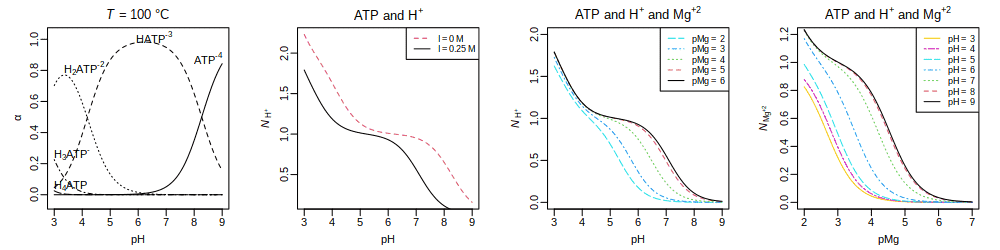Binding of H+ and Mg+2 to ATP at 100 °C and I = 0 M (first plot) or I = 0.25 M (third and fourth plots).

We have calculated the distribution of ATP species and average binding number of H+ and Mg+2 for given pH, pMg, ionic strength, and temperature. Accounting for the distribution of chemical species lends itself to thermodynamic models for reactions between reactants that have multiple ionized and complexed states. In contrast, Alberty (2003) and others propose models for biochemical reactions where the ionized and complexed species are combined into a single representation. Those models invoke Legendre-transformed thermodynamic properties, such as transformed Gibbs energies that are tabulated for specified pH, pMg, and ionic strength. Although the conceptual pathways are different, the two approaches lead to equivalent results concerning the energetics of the overall reactions and the conditions for equilibrium (Sabatini et al., 2012Sabatini A, Vacca A, Iotti S. 2012. Balanced biochemical reactions: A new approach to unify chemical and biochemical thermodynamics. PLOS One 7(1): e29529. doi: 10.1371/journal.pone.0029529). The example here shows how the required calculations can be performed at the species level using conventional standard Gibbs energies for species referenced to infinite dilution (zero ionic strength). The effects of ionic strength are modeled “on the fly” in CHNOSZ by setting the `IS` argument in `subcrt()` or `affinity()` to invoke the nonideality model on top of the standard Gibbs energies of species.

Now that we’re finished, we can reset the nonideality method to the default. (This really isn’t needed here, because there aren’t any nonideality calculations below):

``nonideal(oldnon)``
```## nonideal: setting nonideal option to use Bdot equation
```

# Proteins

Proteins in CHNOSZ are handled a little bit differently from other species. Amino acid group additivity is used to obtain the thermodynamic properties of proteins. Therefore, CHNOSZ has a data file with amino acid compositions of selected proteins, as well as functions for reading and downloading amino acid sequence data.

When proteins in CHNOSZ are identified by name, they include an underscore, such as in `LYSC_CHICK` (chicken lysozyme C). Use `pinfo()` to search for one or more proteins by name; multiple proteins from the same organism can be specified using the `organism` argument. The name search returns the rownumbers of `thermo()\$protein` (i.e. `iprotein`, the protein indices). Supply those protein indices to `pinfo()` to get the amino acid compositions:

``````p1 <- pinfo("LYSC_CHICK")
p2 <- pinfo(c("SHH", "OLIG2"), "HUMAN")
pinfo(c(p1, p2))``````
``````##     protein organism     ref    abbrv chains Ala Cys Asp Glu Phe Gly His Ile
## 6      LYSC    CHICK UniProt   P00698      1  12   8   7   2   3  12   1   6
## 441     SHH    HUMAN UniProt Q15465.N      1  14   3  10  14   5  16   6   7
## 442   OLIG2    HUMAN UniProt   Q13516      1  51   5  10  10   5  35  18   7
##     Lys Leu Met Asn Pro Gln Arg Ser Thr Val Trp Tyr
## 6     6   8   2  14   2   3  11  10   7   6   6   3
## 441  15  11   3   8   7   3  13  12   8   9   3   7
## 442  14  29  11   4  31   5  13  50  11  10   1   3``````

The length and chemical formula of one or more proteins are returned by `protein.length()` and `protein.formula()`. We can calculate the formula of the protein, and the per-residue formula, and show that both have the same average oxidation state of carbon: These functions accept either names or the protein indices (`iprotein`).

``````pl <- protein.length("LYSC_CHICK")
pf <- protein.formula("LYSC_CHICK")
list(length = pl, protein = pf, residue = pf / pl,
ZC_protein = ZC(pf), ZC_residue = ZC(pf / pl))``````
``````## \$length
##  129
##
## \$protein
##              C   H   N   O  S
## LYSC_CHICK 613 959 193 185 10
##
## \$residue
##                  C       H       N       O         S
## LYSC_CHICK 4.75194 7.43411 1.49612 1.43411 0.0775194
##
## \$ZC_protein
##  0.0163132
##
## \$ZC_residue
##  0.0163132``````

The group additivity calculations for proteins are based on equations and data from Amend and Helgeson (2000Amend JP, Helgeson HC. 2000. Calculation of the standard molal thermodynamic properties of aqueous biomolecules at elevated temperatures and pressures. II. Unfolded proteins. Biophysical Chemistry 84(2): 105–136. doi: 10.1016/S0301-4622(00)00116-2), Dick et al. (2006Dick JM, LaRowe DE, Helgeson HC. 2006. Temperature, pressure, and electrochemical constraints on protein speciation: Group additivity calculation of the standard molal thermodynamic properties of ionized unfolded proteins. Biogeosciences 3(3): 311–336. doi: 10.5194/bg-3-311-2006), and LaRowe and Dick (2012LaRowe DE, Dick JM. 2012. Calculation of the standard molal thermodynamic properties of crystalline peptides. Geochimica et Cosmochimica Acta 80: 70–91. doi: 10.1016/j.gca.2011.11.041). There are two major options for the calculations: whether to calculate properties for crystalline or aqueous groups, and, for the latter, whether to model the ionization of the sidechain and terminal groups as a function of pH (and T and P). By default, additivity of aqueous groups is used, but the ionization calculations are not available in `subcrt()`:

``subcrt("LYSC_CHICK")\$out[][1:6, ]``
``````##        T       P      rho    logK         G         H       S       V      Cp
## 1   0.01 1.00000 0.999829 3465.97 -18125553 -44827095 16093.4 10049.2 18448.6
## 2  25.00 1.00000 0.997061 3250.23 -18552315 -44238615 18149.6 10421.0 26842.5
## 3  50.00 1.00000 0.988030 3076.73 -19034574 -43528036 20436.8 10600.2 29597.5
## 4  75.00 1.00000 0.974864 2936.71 -19573864 -42770559 22694.0 10708.1 30863.6
## 5 100.00 1.01322 0.958393 2823.20 -20168495 -41989264 24860.9 10782.9 31582.7
## 6 125.00 2.32014 0.939073 2730.69 -20814620 -41191982 26925.1 10840.9 32071.2``````

Let’s compare experimental values of heat capacity of four proteins, from Privalov and Makhatadze (1990Privalov PL, Makhatadze GI. 1990. Heat capacity of proteins. II. Partial molar heat capacity of the unfolded polypeptide chain of proteins: Protein unfolding effects. Journal of Molecular Biology 213(2): 385–391. doi: 10.1016/S0022-2836(05)80198-6), with those calculated using group additivity. We divide Privalov and Makhatadze’s experimental values by the lengths of the proteins to get per-residue values, then plot them.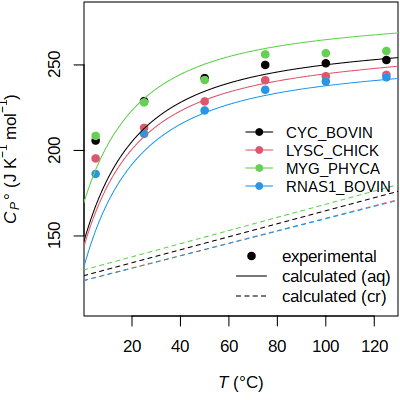The heat capacity calculated by group additivity closely approximates experimental values for aqueous proteins. For a related figure showing the effects of ionization in the calculations, see `?ionize.aa`.

``````PM90 <- read.csv(system.file("extdata/cpetc/PM90.csv", package = "CHNOSZ"))
plength <- protein.length(colnames(PM90)[2:5])
Cp_expt <- t(t(PM90[, 2:5]) / plength)
matplot(PM90[, 1], Cp_expt, type = "p", pch = 19,
xlab = axis.label("T"), ylab = axis.label("Cp0"), ylim = c(110, 280))``````

The loop calculates the properties of each protein using group additivity with aqueous or crystalline groups, then plots the per-residue values.

``````for(i in 1:4) {
pname <- colnames(Cp_expt)[i]
aq <- subcrt(pname, "aq", T = seq(0, 150))\$out[]
cr <- subcrt(pname, "cr", T = seq(0, 150))\$out[]
lines(aq\$T, aq\$Cp / plength[i], col = i)
lines(cr\$T, cr\$Cp / plength[i], col = i, lty = 2)
}
legend("right", legend = colnames(Cp_expt),
col = 1:4, pch = 19, lty = 1, bty = "n", cex = 0.9)
legend("bottomright", legend = c("experimental", "calculated (aq)",
"calculated (cr)"), lty = c(NA, 1, 2), pch = c(19, NA, NA), bty = "n")``````

Although `subcrt()` has no provision for protein ionization, the properties of ionization can be calculated via `affinity()`, which calls `ionize.aa()` if a charged species is in the basis. Whether to calculate properties using aqueous or crystalline groups is determined by the value of `thermo()\$opt\$state`; if it is changed from its default of `aq` to `cr`, no ionization is possible. The following plot shows the calculated affinity of reaction between nonionized proteins and their ionized forms as a function of pH. Charged and uncharged sets of basis species are used to to activate and suppress the ionization calculations. The calculation of affinity for the ionized proteins returns multiple values (as a function of pH), but there is only one value of affinity returned for the nonionized proteins, so we need to use R’s `as.numeric()` to avoid subtracting nonconformable arrays: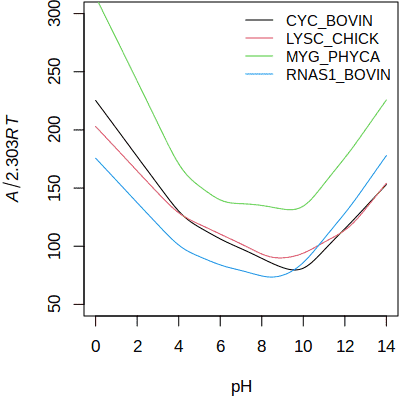Affinity of ionization of proteins. See demo(ionize) for ionization properties calculated as a function of temperature and pH.

``````ip <- pinfo(c("CYC_BOVIN", "LYSC_CHICK", "MYG_PHYCA", "RNAS1_BOVIN"))
basis("CHNOS+")
a_ion <- affinity(pH = c(0, 14), iprotein = ip)
basis("CHNOS")
a_nonion <- affinity(iprotein = ip)
plot(c(0, 14), c(50, 300), xlab = "pH", ylab = quote(italic(A/2.303*RT)), type="n")
for(i in 1:4) {
A_ion <- as.numeric(a_ion\$values[[i]])
A_nonion <- as.numeric(a_nonion\$values[[i]])
lines(a_ion\$vals[], A_ion - A_nonion, col=i)
}
legend("topright", legend = a_ion\$species\$name,
col = 1:4, lty = 1, bty = "n", cex = 0.9)``````

The affinity is always positive, showing the strong energetic drive for ionization of proteins in aqueous solution. The degrees of ionization of amino and carboxyl groups increase at low and high pH, respectively, giving rise to the U-shaped lines.

There, we used the indices returned by `pinfo()` in the `iprotein` argument of `affinity()` to specify the proteins in the calculation. That approach utilizes some optimizations that can be realized due to group additivity, and is useful for calculations involving many proteins. An alternative, but slower, approach is to identify the proteins to `species()`; this produces results that are equivalent to using the `iprotein` argument:

``````species(c("CYC_BOVIN", "LYSC_CHICK", "MYG_PHYCA", "RNAS1_BOVIN"))
a_nonion_species <- affinity()``````
``unlist(a_nonion_species\$values)``
``````##      3450      3449      3453      3455
##   55.5252 -470.1065  228.5332 -495.8844``````

The `ispecies` index (top) refers to the rownumber of `thermo()\$species`; that is distinct from the `iprotein` index (bottom, printed as negative integers), which refers to the rownumber of `thermo()\$protein`.

``unlist(a_nonion\$values)``
``````##        -5        -6        -7        -9
##   52.5252 -473.1065  225.5332 -498.8844``````

## Chemical analysis of proteins

Functions in CHNOSZ make it easy to get the chemical formulas of proteins from their amino acid compositions. Calculations based on the formulas, such as the average oxidation state of carbon (ZC), and the coefficients of basis species in formation reactions, are also available.

Let’s compare the ZC of Rubisco with optimal growth temperature of organisms, as shown in Figure 6a of Dick (2014Dick JM. 2014. Average oxidation state of carbon in proteins. Journal of the Royal Society, Interface 11: 20131095. doi: 10.1098/rsif.2013.1095). First we read a CSV file with the IDs of the proteins and the optimal growth temperatures (Topt); the midpoint of the range of Topt is used for plotting. Then we use `read.fasta()` to read a FASTA file holding the amino acid sequences of the proteins; the function returns a data frame with the amino acid counts. To put the proteins in the right order, the IDs in the CSV file are matched to the names of the proteins in the FASTA file. Then, we calculate ZC from the formulas of the proteins. Next, point symbols are assigned according to domain (Archaea, Bacteria, Eukaryota); numbers inside the symbols show the ordering of Topt in three temperature ranges (0–35 °C, 37.5–60 °C, and 65–100 °C).

Average oxidation state of carbon in Rubisco compared with optimal growth temperature of organisms. This is an interactive image. Move the mouse over the points to show the names of the organisms, and click to open a reference in a new window. (Made with RSVGTipsDevice using code that can be found in the source of this document.)

``````datfile <- system.file("extdata/cpetc/rubisco.csv", package = "CHNOSZ")
fastafile <- system.file("extdata/protein/rubisco.fasta", package = "CHNOSZ")
Topt <- (dat\$T1 + dat\$T2) / 2
idat <- match(dat\$ID, substr(aa\$protein, 4, 9))
aa <- aa[idat, ]
ZC <- ZC(protein.formula(aa))
pch <- match(dat\$domain, c("E", "B", "A")) - 1
col <- match(dat\$domain, c("A", "B", "E")) + 1
plot(Topt, ZC, pch = pch, cex = 2, col = col,
xlab = expression(list(italic(T)[opt], degree*C)),
ylab = expression(italic(Z)[C]))
text(Topt, ZC, rep(1:9, 3), cex = 0.8)
abline(v = c(36, 63), lty = 2, col = "grey")
legend("topright", legend = c("Archaea", "Bacteria", "Eukaryota"),
pch = c(2, 1, 0), col = 2:4, pt.cex = 2)``````

Let’s look at the different ways of representing the chemical compositions of the proteins. `protein.basis()` returns the stoichiometry for the formation reaction of each proteins. Dividing by `protein.length()` gives the per-residue reaction coefficients (n̅). Using the set of basis species we have seen before (CO2, NH3, H2S, H2O, O2) there is a noticeable correlation between n̅O2 and ZC, but even more so between n̅H2O and ZC (shown in the plots on the left). The calculation of ZC, which sums elemental ratios, is not affected by the choice of basis species. The `QEC` keyword to `basis()` loads basis species including three amino acids (glutamine, glutamic acid, cysteine, H2O, O2). This basis strengthens the relationship between ZC and n̅O2, but weakens that between ZC and n̅H2O (shown in the plots on the right).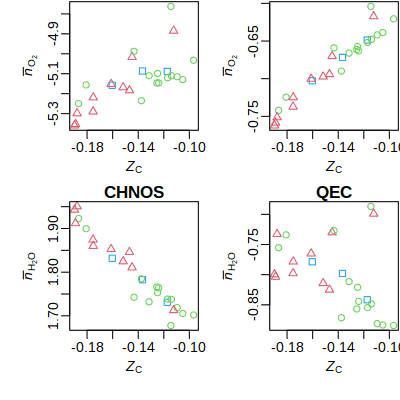Elemental compositions of proteins projected into different sets of basis species.

``````layout(matrix(1:4, nrow = 2))
par(mgp = c(1.8, 0.5, 0))
pl <- protein.length(aa)
ZClab <- axis.label("ZC")
nO2lab <- expression(bar(italic(n))[O])
nH2Olab <- expression(bar(italic(n))[H*O])
lapply(c("CHNOS", "QEC"), function(thisbasis) {
basis(thisbasis)
pb <- protein.basis(aa)
nO2 <- pb[, "O2"] / pl
plot(ZC, nO2, pch = pch, col = col, xlab = ZClab, ylab = nO2lab)
nH2O <- pb[, "H2O"] / pl
plot(ZC, nH2O, pch = pch, col = col, xlab = ZClab, ylab = nH2Olab)
mtext(thisbasis, font = 2)
})``````

By projecting the compositions of proteins into the `QEC` set of basis species, n̅O2 emerges as a strong indicator of oxidation state, while n̅H2O is a relatively uncorrelated (i.e. independent) compositional variable. These independent variables make it easier to distinguish the effects of oxidation and hydration state in proteomic datasets (Dick et al., 2020Dick JM, Yu M, Tan J. 2020. Uncovering chemical signatures of salinity gradients through compositional analysis of protein sequences. Biogeosciences 17(23): 6145–6162. doi: 10.5194/bg-17-6145-2020).

## Normalization to residues

As with other systems, a balance must be chosen for calculations of the metastable equilibrium distribution for proteins. Balancing on the number of backbone units (the sequence length) seems a reasonable choice given the polymeric structure of proteins. Balancing on one of the basis species remains a possibility, using the `balance` argument in `equilibrate()` or `diagram()`. However, there is an additional consideration: owing to the large size of proteins compared to the basis species, the distribution of proteins in metastable equilibrium has many orders of magnitude separation between the activities of the dominant and less-dominant proteins. The metastable coexistence of the residues (i.e. per-residue formulas, or residue equivalents) of the same proteins spans a much smaller range of chemical activities. In CHNOSZ, the calculation of metastable equilibrium activities of the residue equivalents is referred to as normalization.

Let’s look at the metastable equilibrium distribution of selected proteins in the ER-to-Golgi location of S. cerevisiae (yeast) (this example is taken from Dick (2009Dick JM. 2009. Calculation of the relative metastabilities of proteins in subcellular compartments of Saccharomyces cerevisiae. BMC Systems Biology 3: 75. doi: 10.1186/1752-0509-3-75)) Here, we list the names and relative abundances of proteins taken from the YeastGFP study of Ghaemmaghami et al. (2003Ghaemmaghami S, Huh W-K, Bower K, Howson RW, Belle A, Dephoure N, O’Shea EK, Weissman JS. 2003. Global analysis of protein expression in yeast. Nature 425(6959): 737–741. doi: 10.1038/nature02046). There are six proteins identified in the ER-to-Golgi location; one has NA abundance, so it is excluded from the comparisons:

This can be done programmatically using data from the YeastGFP study that are in the JMDplots package:
`y <- JMDplots::yeastgfp(“ER.to.Golgi”)`
`ina <- is.na(y[[“abundance”]])`
`aa <- JMDplots::yeast.aa(y\$protein[!ina])`
`ip <- add.protein(aa)`

``````protein <- c("YDL195W", "YHR098C", "YIL109C", "YLR208W", "YNL049C", "YPL085W")
abundance <- c(1840, 12200, NA, 21400, 1720, 358)
ina <- is.na(abundance)``````

Next, we find the rownumbers of the proteins in `thermo()\$protein`:

``ip <- match(protein[!ina], thermo()\$protein\$protein)``

The YeastGFP study reported absolute abundances of molecules, but the thermodynamic calculations give relative chemical activities of the proteins. In order to make a comparison between them, we use `unitize()` to scale the abundances or activities of proteins (in logarithmic units) such that the total abundance or activity of residue equivalents is unity. To do that, we must have the lengths of the proteins. Here, the first call to `unitize()` generates equal logarithms of activities of proteins for unit total activity of residues; this is used as the reference state for `affinity()`. The second call to `unitize()` scales the logarithms of experimental abundances for unit total activity of residues; this is used for comparison with the theoretical results:

``````pl <- protein.length(ip)
logact <- unitize(numeric(5), pl)
logabundance <- unitize(log10(abundance[!ina]), pl)``````

Now we can load the proteins and calculate their activities in metastable equilibrium as a function of logfO2. The commented line uses `add.OBIGT()` to load group additivity parameters that were present in older versions of CHNOSZ (Dick et al., 2006). The default database contains newer group additivity parameters for the sidechain groups of methionine (LaRowe and Dick, 2012) and glycine and the protein backbone (Kitadai, 2014Kitadai N. 2014. Thermodynamic prediction of glycine polymerization as a function of temperature and pH consistent with experimentally obtained results. Journal of Molecular Evolution 78(3-4): 171–187. doi: 10.1007/s00239-014-9616-1).

``````par(mfrow = c(1, 3))
basis("CHNOS+")
a <- affinity(O2 = c(-80, -73), iprotein = ip, loga.protein = logact)
e <- equilibrate(a)
diagram(e, ylim = c(-5, -2), col = 1:5, lwd = 2)``````

Whoa! The proteins look very non-coexistent in metastable equilibrium. We get a different view by considering per-residue rather than per-protein reactions, through the `normalize` argument for `equilibrate()`: The normalization step is followed by conversion of activities of residues to activities of proteins; that conversion can be skipped using the `as.residue` argument in `equilibrate()`.

``````e <- equilibrate(a, normalize = TRUE)
diagram(e, ylim = c(-5, -2.5), col = 1:5, lwd = 2)
abline(h = logabundance, lty = 1:5, col = 1:5)``````

The experimental relative abundances are plotted as thin horizontal lines with the same style and color as the thicker curved lines calculated for metastable equilibrium. With the exception of YNL049C, the correspondence between the calculations and experiments looks to be greatest near the middle-left part of the figure.

ER-to-Golgi proteins: calculations without and with length normalization.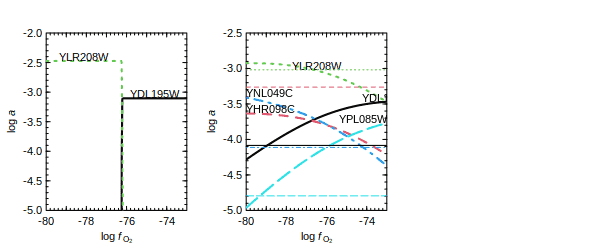## Getting amino acid compositions

In the Rubisco example above, we saw the use of `read.fasta()` to read amino acid sequences from a FASTA file. There are several other methods for inputting amino acid compositions.

R’s `read.csv()` can be used to read amino acid compositions from a CSV file with the same columns that are present in `thermo()\$protein`. Note the use of `as.is = TRUE` to prevent reading character data as factors. The `nrows` argument can be added to read that number of rows:

``````file <- system.file("extdata/protein/POLG.csv", package = "CHNOSZ")
aa_POLG <- read.csv(file, as.is = TRUE, nrows = 5)``````

`read.fasta()` reads a FASTA file and returns the amino acid compositions of the sequences. The `iseq` argument can be used to read those sequences from the file:

``````file <- system.file("extdata/protein/EF-Tu.aln", package = "CHNOSZ")
aa_Ef <- read.fasta(file, iseq = 1:2)``````

`seq2aa()` counts the amino acids in a user-supplied sequence and generates a data frame of the amino acid composition: See also `?count.aa`, which can process both protein and nucleic acid sequences.

``````aa_PRIO <- seq2aa("
MANLGCWMLVLFVATWSDLGLCKKRPKPGGWNTGGSRYPGQGSPGGNRYPPQGGGGWGQP
HGGGWGQPHGGGWGQPHGGGWGQPHGGGWGQGGGTHSQWNKPSKPKTNMKHMAGAAAAGA
VVGGLGGYMLGSAMSRPIIHFGSDYEDRYYRENMHRYPNQVYYRPMDEYSNQNNFVHDCV
NITIKQHTVTTTTKGENFTETDVKMMERVVEQMCITQYERESQAYYQRGSSMVLFSSPPV
ILLISFLIFLIVG
", "PRIO_HUMAN")``````

These amino acid compositions can be processed using functions such as `protein.length()` and `protein.formula()`:

``````myaa <- rbind(aa_Ef, aa_PRIO)
protein.length(myaa)``````
``##  394 394 253``

# Sources of thermodynamic data

An attempt has been made to assemble a default database that has no major inconsistencies between species. As the database includes thermodynamic data from many sources, it can not be guaranteed to be fully internally consistent. For crucial problems, check not only the accuracy of entries in the database, but also the suitability of the data for your problem. If there are any doubts, consult the primary sources.

Most of the species in OBIGT have parameters for one of two models for calculating thermodynamic properties. The coefficients in these models are indicated by the column names with a dot, for example `a1.a`. Most aqueous species use the revised Helgeson-Kirkham-Flowers (HKF) equations (text before the dot), and crystalline, gas and liquid species other than H2O use a polynomial equation for heat capacity. See `?hkf` and `?cgl` for information about the equations used for thermodynamic properties.

• Be sure to read the help page at `?thermo` for information about the format of the OBIGT data frame.
• See the vignette OBIGT thermodynamic database for a summary of all sources for the default database and optional data files.
• See the vignette Customizing the thermodynamic database for a broader description of the structure of OBIGT, data entry conventions, and examples of adding species to the database.

## Viewing data sources: `thermo.refs()`

The OBIGT database lists one or two sources for each entry, and citation information for the sources is listed in `thermo()\$refs`. You can locate and view the references with `thermo.refs()`. Running the function without any arguments opens a browser window with the complete table of references. See the vignette OBIGT thermodynamic database for a more nicely formatted presentation of the sources of thermodynamic data, along with notes and additional comments. Where available, links to the web page for the articles and books are displayed:

``thermo.refs()  ## shows table in a browser``

A numeric argument to `thermo.refs()` gives one or more species indices for which to get the references:

``````iATP <- info("ATP-4")
iMgATP <- info("MgATP-2")
thermo.refs(c(iATP, iMgATP))``````
``````##       key                          author year                               citation                                                    note
## 149 LH06a D. E. LaRowe and H. C. Helgeson 2006 Geochim. Cosmochim. Acta 70, 4680-4724        nucleic-acid bases, nucleosides, and nucleotides
## 151 LH06b D. E. LaRowe and H. C. Helgeson 2006           Thermochim. Acta 448, 82-106 Mg-complexed adenosine nucleotides (ATP), NAD, and NADP
##                                           URL
## 149 https://doi.org/10.1016/j.gca.2006.04.010
## 151 https://doi.org/10.1016/j.tca.2006.06.008``````

A character argument gives the source key(s):

``thermo.refs(c("HDNB78", "MGN03"))``
``````##        key                                    author year                 citation                                       note                                    URL
## 7   HDNB78       H. C. Helgeson, J. M. Delany et al. 1978  Am. J. Sci. 278A, 1-229    data for minerals and phase transitions https://www.worldcat.org/oclc/13594862
## 135  MGN03 J. Majzlan, K.-D. Grevel and A. Navrotsky 2003 Am. Mineral. 88, 855-859 goethite, lepidocrocite, and maghemite GHS  https://doi.org/10.2138/am-2003-5-614``````

If the argument holds the result of `subcrt()`, references for all species in the reaction are returned: The exception is H2O. With the default settings, thermodynamic properties for H2O are derived from SUPCRT92 (Johnson et al., 1992).

``````sres <- subcrt(c("C2H5OH", "O2", "CO2", "H2O"), c(-1, -3, 2, 3))
thermo.refs(sres)``````
``````##        key                                             author year                               citation                                                 note
## 124   PS01                    A. V. Plyasunov and E. L. Shock 2001 Geochim. Cosmochim. Acta 65, 3879-3900            aqueous nonelectrolytes (organic species)
## 32   SHS89   E. L. Shock, H. C. Helgeson and D. A. Sverjensky 1989 Geochim. Cosmochim. Acta 53, 2157-2183                            inorganic neutral species
## 125 PS01.1                    A. V. Plyasunov and E. L. Shock 2001 Geochim. Cosmochim. Acta 65, 3879-3900 aqueous nonelectrolytes (Ar, Xe, and CO<sub>2</sub>)
## 18   HGK84         L. Haar and J. S. Gallagher and G. S. Kell 1984                   NBS/NRC Steam Tables                                       H<sub>2</sub>O
## 45   JOH92 J. W. Johnson and E. H. Oelkers and H. C. Helgeson 1992              Comp. Geosci. 18, 899-947                                       H<sub>2</sub>O
##                                               URL
## 124 https://doi.org/10.1016/S0016-7037(01)00678-0
## 32   https://doi.org/10.1016/0016-7037(89)90341-4
## 125 https://doi.org/10.1016/S0016-7037(01)00678-0
## 18        https://www.worldcat.org/oclc/858456124
## 45   https://doi.org/10.1016/0098-3004(92)90029-Q``````

The URLs of the references can be copied to a browser, or opened using R’s `browseURL()`:

``````iFo <- info("forsterite")
ref <- thermo.refs(iFo)
browseURL(ref\$URL)  ## opens a link to worldcat.org``````

## Default database

Thermodynamic properties of minerals in the default database are mostly taken from Berman (1988Berman RG. 1988. Internally-consistent thermodynamic data for minerals in the system Na2O–K2O–CaO–MgO–FeO–Fe2O3–Al2O3–SiO2–TiO2–H2O–CO2. Journal of Petrology 29(2): 445–522. doi: 10.1093/petrology/29.2.445) (including silicates, aluminosilicates, calcite, dolomite, hematite, and magnetite) and Helgeson et al. (1978Helgeson HC, Delany JM, Nesbitt HW, Bird DK. 1978. Summary and critique of the thermodynamic properties of rock-forming minerals. American Journal of Science 278A: 1–229. Available at https://www.worldcat.org/oclc/13594862. ) (native elements, sulfides, halides, sulfates, and selected carbonates and oxides that do not duplicate any in the Berman dataset). Minerals are identified by the state `cr`, and (for the Helgeson dataset) `cr2`, `cr3`, etc. for higher-temperature polymorphs.

Compared to SUPCRT92/SLOP98 (see below), the default database include updates for aqueous Al species (Tagirov and Schott, 2001Tagirov B, Schott J. 2001. Aluminum speciation in crustal fluids revisited. Geochimica et Cosmochimica Acta 65(21): 3965–3992. doi: 10.1016/S0016-7037(01)00705-0), Au species (Pokrovski et al., 2014Pokrovski GS, Akinfiev NN, Borisova AY, Zotov AV, Kouzmanov K. 2014. Gold speciation and transport in geological fluids: Insights from experiments and physical-chemical modelling. Geological Society, London, Special Publications 402(1): 9–70. doi: 10.1144/SP402.4) (see `demo(gold)`), and arsenic-bearing aqueous species and minerals, as compiled in the SUPCRTBL package (Zimmer et al., 2016Zimmer K, Zhang Y, Lu P, Chen Y, Zhang G, Dalkilic M, Zhu C. 2016. SUPCRTBL: A revised and extended thermodynamic dataset and software package of SUPCRT92. Computers & Geosciences 90: 97–111. doi: 10.1016/j.cageo.2016.02.013). This list of updates is incomplete; see the vignette OBIGT thermodynamic database for a detailed list of data sources.

## Optional data files

Some optional datasets can be activated by using `add.OBIGT()`. The first couple of these contain data that have been replaced by or are incompatible with later updates; the superseded data are kept here to reproduce published calculations and for comparison with the newer data:

`add.OBIGT("SUPCRT92")` – This file contains data for minerals from SUPCRT92 (mostly Helgeson et al., 1978) that have been replaced by the Berman data set.

`add.OBIGT("SLOP98")` – This file contains data from `slop98.dat` or later slop files, from Everett Shock’s GEOPIG group at Arizona State University, that were previously used in CHNOSZ but have been replaced by more recent data updates. Some calculations using the older data are shown in this vignette.

The updates for these data have been taken from various publications (LaRowe and Dick, 2012; Kitadai, 2014; Azadi et al., 2019) A comparison of logK of metal-glycinate complexes using the updated data is in `demo(glycinate)`.

The next three optional datasets are provided to support newer data or models:

`add.OBIGT("AD")` – These data are used in the Akinfiev-Diamond model for aqeuous nonelectrolytes (Akinfiev and Diamond, 2003Akinfiev NN, Diamond LW. 2003. Thermodynamic description of aqueous nonelectrolytes at infinite dilution over a wide range of state parameters. Geochimica et Cosmochimica Acta 67(4): 613–629. doi: 10.1016/S0016-7037(02)01141-9).

`add.OBIGT("AS04")` – This file has data for aqueous SiO2 from Apps and Spycher (2004Apps J, Spycher N. 2004. Data qualification for thermodynamic data used to support THC calculations. Las Vegas, NV: Bechtel SAIC Company, LLC. Report No.: ANL-NBS-HS-000043 REV 00 (DOC.20041118.0004).) and modified HSiO3-. These data are included to help transition to the “higher solubility of quartz paradigm” (Wolery and Jové Colón, 2017Wolery TJ, Jové Colón CF. 2017. Chemical thermodynamic data. 1. The concept of links to the chemical elements and the historical development of key thermodynamic data. Geochimica et Cosmochimica Acta 213(Supplement C): 635–676. doi: 10.1016/j.gca.2016.09.028), but are not included in the default database in order to maintain compatibility with existing data for minerals that are linked to the older aqueous SiO2 data. See `demo(aluminum)` for an example.

`add.OBIGT("DEW")` – These are aqueous species, with modified parameters, that are intended for use with the Deep Earth Water (DEW) model (Sverjensky et al., 2014Sverjensky DA, Harrison B, Azzolini D. 2014. Water in the deep Earth: The dielectric constant and the solubilities of quartz and corundum to 60 kb and 1,200 °C. Geochimica et Cosmochimica Acta 129: 125–145. doi: 10.1016/j.gca.2013.12.019). You should also run `water("DEW")` to activate the equations in the model; then, they will be used by `subcrt()` and `affinity()`. Examples are in `demo(DEW)`.

`add.OBIGT("GEMSFIT")` – Thermodynamic data for aqueous species in the system Ca-Mg-Na-K-Al-Si-O-H-C-Cl obtained from global optimization of Gibbs energies with the GEMSFIT package (Miron et al., 2017Miron GD, Wagner T, Kulik DA, Lothenbach B. 2017. An internally consistent thermodynamic dataset for aqueous species in the system Ca-Mg-Na-K-Al-Si-O-H-C-Cl to 800 °C and 5 kbar. American Journal of Science 317(7): 755–806. doi: 10.2475/07.2017.01).

## Cross-checking data entries

`info()` automatically performs some cross-checks of the thermodynamic data. This only checks the parameters for individual species, not the internal consistency of the database itself. Given a numeric species index, it runs `checkEOS()`, which is a function that calculates values of CP° and V° from the HKF or heat capacity parameters and indicates whether differences from the database are greater than 1 cal K-1 mol-1 or 1 cm3 mol-1. `info()` also runs `checkGHS()`, which calculates the value of ΔG°f from those of ΔH°f and S° and from the entropy of the elements (Cox et al., 1989Cox JD, Wagman DD, Medvedev VA, editors. 1989. CODATA Key Values for Thermodynamics. New York: Hemisphere Publishing Corporation. Available at https://www.worldcat.org/oclc/18559968. ) in the chemical formula of the species.

Some species in the default and optional databases are known to have inconsistent parameters. For instance, we can check the data for the trisulfur radical ion (S3-) from Pokrovski and Dubessy (2015Pokrovski GS, Dubessy J. 2015. Stability and abundance of the trisulfur radical ion in hydrothermal fluids. Earth and Planetary Science Letters 411: 298–309. doi: 10.1016/j.epsl.2014.11.035):

``info(info("S3-"))``
```## checkGHS: G of S3-(aq) differs by 661 cal mol-1 from tabulated value
```

The calculated value of ΔG°f is 661 cal mol-1 higher than the entered value. Because the difference between the entered and calculated values of ΔG°f is greater than 100 cal mol-1, `checkGHS()` produces a message. After checking for any typographical errors in the entries for ΔG°f, ΔH°f, S°, and the chemical formula, the source should be consulted for clarification.

All of the species with inconsistencies detected in this manner, in both OBIGT and the optional data files, are listed in the file `OBIGT_check.csv`.

``````file <- system.file("extdata/adds/OBIGT_check.csv", package = "CHNOSZ")
dat <- read.csv(file, as.is = TRUE)
nrow(dat)``````
``##  363``

Without additional information, there is often no clear strategy for “fixing” these inconsistent data, and they are provided as-is. However, users are encouraged to send any corrections they find to the package maintainer.

## Water: SUPCRT92 or IAPWS-95 or DEW

For calculations of the thermodynamic and dielectric properties of liquid and supercritical H2O, CHNOSZ uses a Fortran subroutine (`H2O92`) from SUPCRT92 (Johnson et al., 1992). Alternatively, the IAPWS-95 formulation for thermodynamic properties (Wagner and Pruß, 2002Wagner W, Pruß A. 2002. The IAPWS formulation 1995 for the thermodynamic properties of ordinary water substance for general and scientific use. Journal of Physical and Chemical Reference Data 31(2): 387–535. doi: 10.1063/1.1461829) can be utilized. In part because of intrinsic thermodynamic differences between SUPCRT92 and IAPWS-95, as well as different equations used in CHNOSZ for calculating the dielectric constant when the IAPWS-95 option is active, this option could introduce inconsistencies with the data for aqueous species in the database and is not recommended for general use in CHNOSZ. However, the IAPWS-95 equations are useful for other applications, and may be extrapolated to a greater range of T and P than SUPCRT. See `?water` for more information, as well as the last example in `?subcrt`, where uncommenting the line for the `IAPWS95` option allows extrapolation to lower temperatures for supercooled water.

An implementation of the Deep Earth Water (DEW) model is also available; see Optional data for more information.

# Functions outside the main workflow

Some functions in CHNOSZ lie outside the main workflow described above.

• `RH2OBIGT()` implements a group additivity calculation of standard molal thermodynamic properties and equations of state parameters of crystalline and liquid organic molecules from Richard and Helgeson (1998Richard L, Helgeson HC. 1998. Calculation of the thermodynamic properties at elevated temperatures and pressures of saturated and aromatic high molecular weight solid and liquid hydrocarbons in kerogen, bitumen, petroleum, and other organic matter of biogeochemical interest. Geochimica et Cosmochimica Acta 62(23-24): 3591–3636. doi: 10.1016/S0016-7037(97)00345-1).

• Some functions are available (see `?taxonomy`) to read data from NCBI taxonomy files, traverse taxonomic ranks, and get scientific names of taxonomic nodes.

# Citation and contact information

To cite CHNOSZ, use this reference:

Dick JM (2019). “CHNOSZ: Thermodynamic calculations and diagrams for geochemistry.” Frontiers in Earth Science, 7, 180. doi:10.3389/feart.2019.00180.

For the features described in the multi-metal vignette, use this reference:

Dick JM (2021). “Diagrams with multiple metals in CHNOSZ.” Applied Computing and Geosciences, 10, 100059. doi:10.1016/j.acags.2021.100059.

``maintainer("CHNOSZ")``
``##  "Jeffrey Dick <j3ffdick@gmail.com>"``

Thank you for reading, and have fun!

“The real fun of life is this perpetual testing to realize how far out you can go with any potentialities.”

# Document history

View the R Markdown source of this document on R-Forge or in R:

``file.edit(system.file("doc/anintro.Rmd", package = "CHNOSZ"))``

``````   ######    ##   ##    ##   ##    ######     #####  #####
##         ##===##    ## \\##   ##    ##     \\       //
######    ##   ##    ##   ##    ######    #####      #####``````